# Using weights in regression examples

I have come across several different examples recently where ‘use weights in regression’ was the solution to a particular problem. I will outline four recent examples.

# Example 1: Rates in WDD

Sophie Curtis-Ham asks whether I can extend my WDD rate example to using the Poisson regression approach I outline. I spent some time and figured out the answer is yes.

First, if you install my R package ptools, we can use the same example in that blog post showing rates (or as per area, e.g. density) in my internal `wdd` function using R code (Wheeler & Ratcliffe, 2018):

``````library(ptools)

crime <- c(207,308,178,150,110,318,157,140)
type <- c('t','ct','d','cd','t','ct','d','cd')
ti <- c(0,0,0,0,1,1,1,1)
ar <- c(1.2,0.9,1.5,1.6,1.2,0.9,1.5,1.6)

df <- data.frame(crime,type,ti,ar)

# The order of my arguments is different than the
# dataframe setup, hence the c() selections
weight_wdd <- wdd(control=crime[c(2,6)],
treated=crime[c(1,5)],
disp_control=crime[c(4,8)],
disp_treated=crime[c(3,7)],
area_weights=ar[c(2,1,4,3)])

# Estimate -91.9 (31.5) for local``````

So here the `ar` vector is a set of areas (imagine square miles or square kilometers) for treated/control/displacement/displacementcontrol areas. But it would work the same if you wanted to do person per-capita rates as well.

Note that the note says the estimate for the local effect, in the glm I will show I am just estimating the local, not the displacement effect. At first I tried using an offset, and that did not change the estimate at all:

``````# Lets do a simpler example with no displacement
df_nod <- df[c(1,2,5,6),]
df_nod['treat'] <- c(1,0,1,0)
df_nod['post'] <- df_nod['ti']

# Attempt 1, using offset
m1 <- glm(crime ~ post + treat + post*treat + offset(log(ar)),
data=df_nod,
summary(m1) # estimate is  -107 (30.7), same as no weights WDD``````

Maybe to get the correct estimate via the offset approach you need to do some post-hoc weighting, I don’t know. But we can use weights and estimate the rate on the left hand side.

``````# Attempt 2, estimate rate and use weights
# suppressWarnings is for non-integer notes
df_nod['rate'] <- df_nod['crime']/df_nod['ar']
m2 <- suppressWarnings(glm(rate ~ post + treat + post*treat,
data=df_nod,
weights=ar,
summary(m2) # estimate is same as no weights WDD, -91.9 (31.5)``````

The motivation again for the regression approach is to extend the WDD test to scenarios more complicated than simple pre/post, and using rates (e.g. per population or per area) seems to be a pretty simple thing people may want to do!

# Example 2: Clustering of Observations

Had a bit of a disagreement at work the other day – statistical models used for inference of coefficients on the right hand side often make the “IID” assumption – independent and identically distributed residuals (or independent observations conditional on the model). This is almost entirely focused on standard errors for right hand side coefficients, when using machine learning models for purely prediction it may not matter at all.

Even if interested in inference, it may be the solution is to simply weight the regression. Consider the most extreme case, we simply double count (or here repeat count observations 100 times over):

``````# Simulating simple Poisson model
# but replicating data
set.seed(10)
n <- 600
repn <- 100
id <- 1:n
x <- runif(n)
l <- 0.5 + 0.3*x
y <- rpois(n,l)
small_df <- data.frame(y,x,id)
big_df <- data.frame(y=rep(y,repn),x=rep(x,repn),id=rep(id,repn))

# With small data
mpc <- glm(y ~ x, data=small_df, family=poisson)
summary(mpc)

# Note same coefficients, just SE are too small
mpa <- glm(y ~ x, data=big_df, family=poisson)

vcov(mpc)/vcov(mpa) # ~ 100 times too small``````

So as expected, the standard errors are 100 times too small. Again this does not cause bias in the equation (and so will not cause bias if the equation is used for predictions). But if you are making inferences for coefficients on the right hand side, this suggests you have way more precision in your estimates than you do in reality. One solution is to simply weight the observations inverse the number of repeats they have:

``````big_df\$w <- 1/repn
mpw <- glm(y ~ x, weight=w, data=big_df, family=poisson)
summary(mpw)
vcov(mpc)/vcov(mpw) # correct covariance estimates``````

And this will be conservative in many circumstances, if you don’t have perfect replication across observations. Another approach though is to cluster your standard errors, which uses data to estimate the residual autocorrelation inside of your groups.

``````library(sandwich)
vcov(mpc)/adj_mpa   # much closer, still *slightly* too small``````

I use HC2 here as it uses small sample degree of freedom corrections (Long & Ervin, 2000). There are quite a few different types of cluster corrections. In my simulations HC2 tends to be the “right” choice (likely due to the degree of freedom correction), but I don’t know if that should generally be the default for clustered data, so caveat emptor.

Note again though that the cluster standard error adjustments don’t change the point estimates at all – they simply adjust the covariance matrix estimates for the coefficients on the right hand side.

# Example 3: What estimate do you want?

So in the above example, I exactly repeated everyone 100 times. You may have scenarios where you have some observations repeated more times than others. So above if I had one observation repeated 10 times, and another repeated 2 times, the correct weights in that scenario would be 1/10 and 1/2 for each row inside the clusters/repeats. Here is another scenario though where we want to weight up repeat observations though – it just depends on the exact estimate you want.

A questioner wrote in with an example of a discrete choice type set up, but some respondents are repeated in the data (e.g. chose multiple responses). So imagine we have data:

``````Person,Choice
1      A
1      B
1      C
2      A
3      B
4      B  ``````

If you want to know the estimate in this data, “pick a random person-choice, what is the probability of choosing A/B/C?”, the answer is:

``````A - 2/6
B - 3/6
C - 1/6``````

But that may not be what you really want, it may be you want “pick a random person, what is the probability that they choose A/B/C?”, so in that scenario the correct estimate would be:

``````A - 2/4
B - 3/4
C - 1/4``````

To get this estimate, we should weight up responses! So typically each row would get a weight of 1/nrows, but here we want the weight to be 1/npersons and constant across the dataset.

``````Person,Choice,OriginalWeight,UpdateWeight
1      A      1/6             1/4
1      B      1/6             1/4
1      C      1/6             1/4
2      A      1/6             1/4
3      B      1/6             1/4
4      B      1/6             1/4``````

And this extends to whatever regression model if you want to model the choices as a function of additional covariates. So here technically person 1 gets triple the weight of persons 2/3/4, but that is the intended behavior if we want the estimate to be “pick a random person”.

Depending on the scenario you could do two models – one to estimate the number of choices and another to estimate the probability of a specific choice, but most people I imagine are not using such models for predictions so much as they are for inferences on the right hand side (e.g. what influences your choices).

# Example 4: Cross-classified data

The last example has to do with observations that are nested within multiple hierarchical groups. One example that comes up in spatial criminology – we want to do analysis of some crime reduction/increase in a buffer around a point of interest, but multiple buffers overlap. A solution is to weight observations by the number of groups they overlap.

For example consider converting incandescent street lamps to LED (Kaplan & Chalfin, 2021). Imagine that we have four street lamps, {c1,c2,t1,t2}. The figure below display these four street lamps; the t street lamps are treated, and the c street lamps are controls. Red plus symbols denote crime locations, and each street lamp has a buffer of 1000 feet. The two not treated circle street lamps overlap, and subsequently a simple buffer would double-count crimes that fall within both of their boundaries.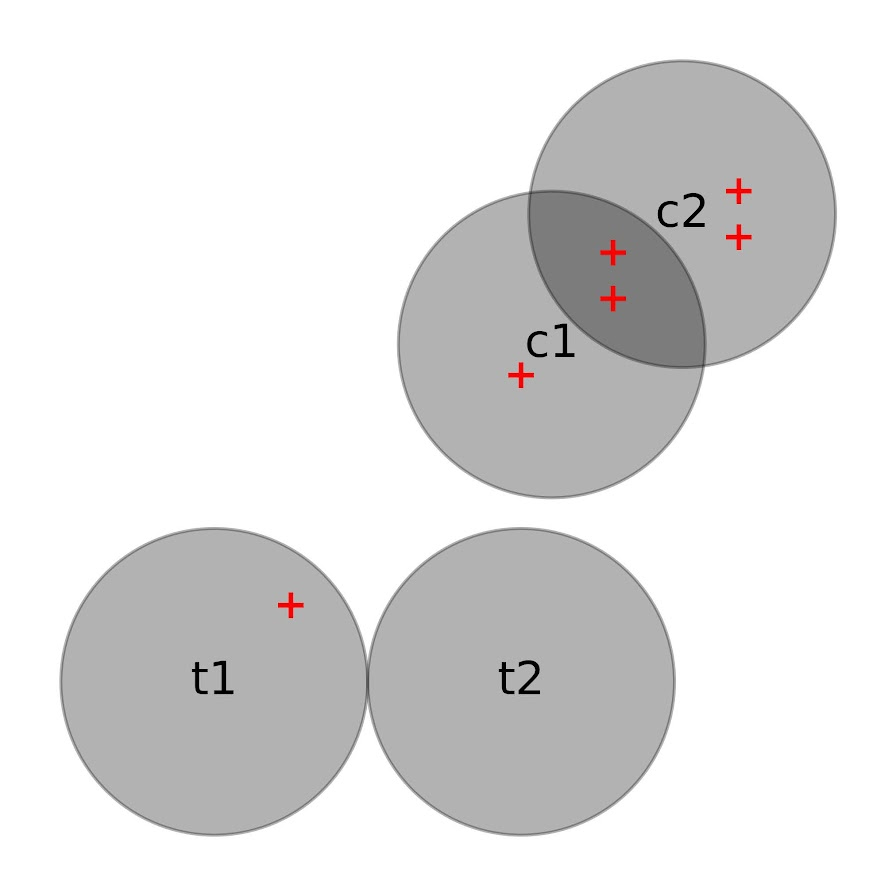If one estimated a treatment effect based on these buffer counts, with the naive count within buffer approach, one would have:

``````c1 = 3    t1 = 1
c2 = 4    t2 = 0``````

Subsequently an average control would then be 3.5, and the average treated would be 0.5. Subsequently one would have an average treatment effect of 3. This however would be an overestimate due to the overlapping buffers for the control locations. Similar to example 3 it depends on how exactly you want to define the average treatment effect – I think a reasonable definition is simply the global estimate of crimes reduced divided by the total number of treated areas.

To account for this, you can weight individual crimes. Those crimes that are assigned to multiple street lamps only get partial weight – if they overlap two street lamps, the crimes are only given a weight of 0.5, if they overlap three street lamps within a buffer area those crimes are given a weight of 1/3, etc. With such updated weighted crime estimates, one would then have:

``````c1 = 2    t1 = 1
c2 = 3    t2 = 0``````

And then one would have an average of 2.5 crimes in the control street lamps, and subsequently would have a treatment effect reduction per average street lamp of 2 crimes overall.

This idea I first saw in Snijders & Bosker (2011), in which they called this cross-classified data. I additionally used this technique with survey data in Wheeler et al. (2020), in which I nested responses in census tracts. Because responses were mapped to intersections, they technically could be inside multiple census tracts (or more specifically I did not know 100% what tract they were in). I talk about this issue in my dissertation a bit with crime data, see pages 90-92 (Wheeler, 2015). In my dissertation using D.C. data, if you aggregated that data to block groups/tracts the misallocation error is likely ~5% in the best case scenario (and depending on data and grouping, could be closer to 50%).

But again I think a reasonable solution is to weight observations, which is not much different to Hipp & Boessan’s (2013) egohoods.

# Fitting a plateau effects model in scipy

Dealing with a few models recently that people fit non-linear effects (either via polynomials or splines), and the results are just on their face too curvy.

There is also a common social science trope where people fit a polynomial to some data, and that clearly exploratory model fitting exercise becomes a main focal point of the paper.

But there is one scenario I commonly see though for curves that I think makes sense for quite a bit of social science data – a plateau effect. See for example this Hipp article that finds a plateau effect for poverty -> crime rates. John though uses a cubic function later to fit these effects, so it curves back down – I think a more reasonable model would enforce monotonic constraints so it doesn’t dip back down in the tails of the data. (The same issue often happens with quadratic polynomials as well.) I have some other blog posts on segmented models as well that are subject to the same not being monotonic where they should be.

A plateau model is difficult to fit out of the box though in most current stat software. Rick Wicklin on his blog has a nice formulation though: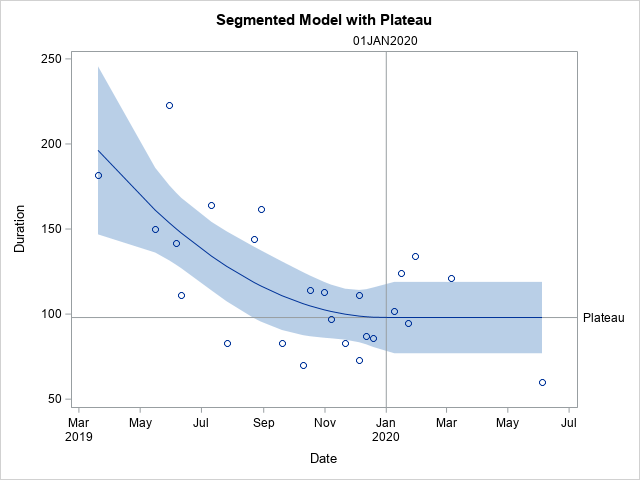It fits a quadratic, and then plateaus after a particular breakpoint. For theory testing I imagine the breakpoint itself will be of interest to many criminologists, and you can estimate that location in this formulation.

Rick works for SAS, and so if familiar with SAS go ahead and use his code. But here I coded up an example fitting a constrained non-linear regression in python using scipy.

# Python Code

Taking the same data from Rick Wicklin’s blog post, this code just reads in the data and converts dates to days since 3/20/2019. I don’t scale the data here to be an exact replicate of Rick’s blog post, but for data with a wider range it would be necessary to prevent some numerical instability.

``````# Python libraries to replicate

from datetime import datetime
import numpy as np
import pandas as pd
from scipy.optimize import minimize
from scipy.optimize import NonlinearConstraint

# Via https://blogs.sas.com/content/iml/2020/12/14/segmented-regression-sas.html
dat = [(1,'3/20/2019',182),
(3,'5/30/2019',223),
(5,'6/11/2019',111),
(7,'7/26/2019',83),
(9,'8/29/2019',162),
(11,'10/10/2019',70),
(13,'10/31/2019',113),
(15,'11/21/2019',83),
(17,'12/5/2019',73),
(19,'12/19/2019',86),
(21,'1/16/2020',124),
(23,'1/30/2020',134),
(25,'6/4/2020',60),
(2,'5/16/2019',150),
(4,'6/6/2019',142),
(6,'7/11/2019',164),
(8,'8/22/2019',144),
(10,'9/19/2019',83),
(12,'10/17/2019',114),
(14,'11/7/2019',97),
(16,'12/5/2019',111),
(18,'12/12/2019',87),
(20,'1/9/2020',102),
(22,'1/23/2020',95),
(24,'3/5/2020',121)]

df = pd.DataFrame(dat,columns=['SugeryNo','Date','Duration'])
df['Date'] = pd.to_datetime(df['Date'])
df['DaysRef'] = (df['Date'] - pd.to_datetime('3/20/2019')).dt.days
df['DR2'] = df['DaysRef']**2``````

Now, one of the things I sometimes find confusing in posts that optimize arbitrary functions (in R or python) is that to minimize a function, it is with respect to your data at hand. Sometimes folks have functions that can pass in data and the parameters. But I find it easier to just keep the data fixed and only pass in parameters.

So you can see in my non-linear pred function, it passes in the parameters (which we will estimate), and gives a prediction for the fixed dataset. Ditto for the loss function (you could update to do logistic regression for example if predicting 0/1s). Then the `nlconst` object is a special python function to define the non-linear constraints that make this plateau model work. Then start solutions and finally minimize the function (using a Fortran solver!):

``````# Pass in global data into the function
def prednl(x):
b0 = x
b1 = x
b2 = x
brp = x
before = (df['DaysRef'] < brp)
y0 = b0 + b1*df['DaysRef'] + b2*df['DR2']
y1 = b0 + b1*brp + b2*brp*brp
return y0*before + (~before)*y1

def lossnl(x):
yhat = prednl(x)
squares = (df['Duration'] - yhat)**2
return squares.sum()

def nlconst(x):
r1 = x - (x + x*x + x*x*x)    # plateau
r2 = x - ((-0.5*x)/x)                     # breakpoint
# Could also consider bounds on breakpoint and curve needs to be non-zero
return np.array([r1,r2])

nlc = NonlinearConstraint(nlconst, np.array([0.0,0.0]),
np.array([0.0,0.0]))

start = np.array([185.0,-1.0,0.1,150.0,60.0])

solution = minimize(lossnl,start,method='trust-constr',
constraints=nlc,options={'maxiter':50000})``````

And this returns the same fit as did the SAS routine: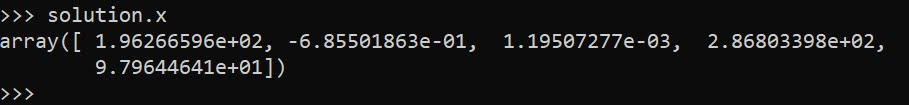Now I will admit defeat to trying to figure out analytical standard errors (tried via the outer product gradient approach via `autograd`, as well as using BFGS and its inverse hessian estimate, which is not even close to the results SAS gives).

So I do the thing all lazy statisticians do at this point – the bootstrap. (SPSS I believe will only give standard errors for its nonlinear estimates via bootstrap.)

``````# Do the bootstrap, 95% CI
res = []
mess = []
for i in range(19):
print(f'iter {i+1}: ',datetime.now())
boot = df.sample(n=df.shape,replace=True).reset_index(drop=True)
days_ref = boot['DaysRef'].to_numpy()
duration = boot['Duration'].to_numpy()
dr2 = boot['DR2'].to_numpy()
def lb(x):
b0 = x
b1 = x
b2 = x
brp = x
before = (days_ref < brp)
y0 = b0 + b1*days_ref + b2*dr2
y1 = b0 + b1*brp + b2*brp*brp
yhat = y0*before + (~before)*y1
squares = (duration - yhat)**2
return squares.sum()
sl = minimize(lb,start,method='trust-constr',
constraints=nlc,options={'maxiter':50000})
mess.append(sl.message)
print(sl.message)
res.append(sl.x)

rdf = pd.DataFrame(res,columns=['B0','B1','B2','break','plateau'])
rdf.describe() #min/max are the 95% CIs``````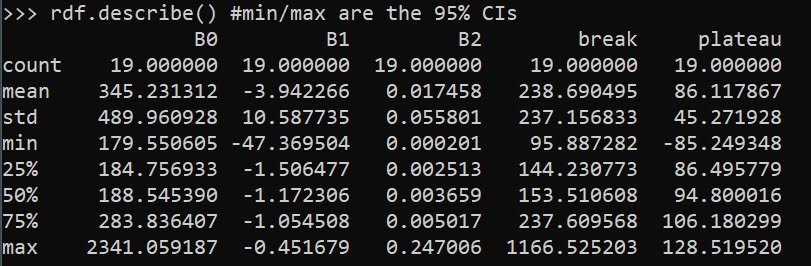And we can see that these estimates are very wide. We can look at individual iterations, and in a few the estimates go off the rails (and they still say they converged, they just converged to non-sense).

``````# Some of the wayward estimates
# still pass convergence
rdf['Eval'] = mess
rdf``````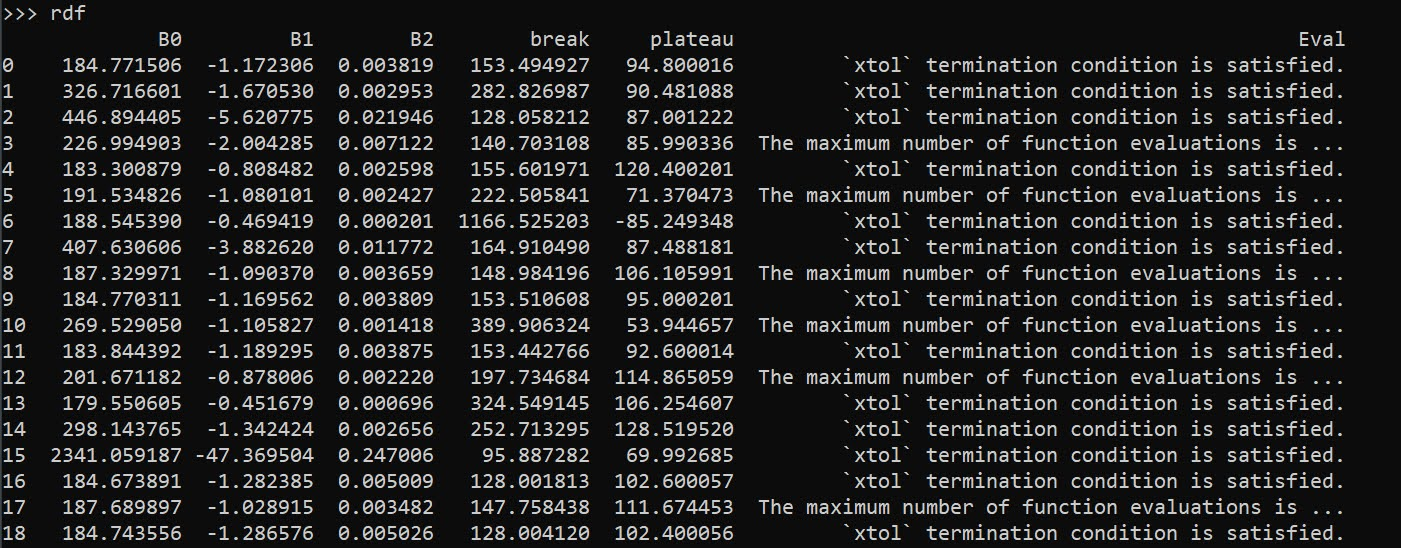But this is the nature of these non-linear functions. They can be pretty finicky. If a straight line fits the data quite well, the quadratic term will be very small, and so the estimated plateau may be outside of the data (or just totally unstable).

Still, even though it is more work and potentially more finicky in model fitting, I would rather people have explicit functional form predictions for non-linear effects, than simply throwing in polynomial functions and writing a paper about “look at these non-linear effects”.

And this formulation provides an explicit mechanism to measure the location of a plateau effect directly as a parameter.

# Wald tests via statsmodels (python)

The other day on crossvalidated a question came up about interpreting treatment effect differences across different crime types. This comes up all the time in criminology research, especially interventions intended to reduce crime.

Often times interventions are general and may be expected to reduce multiple crime types, e.g. hot spots policing may reduce both violent crimes and property crimes. But we do not know for sure – so it makes sense to fit models to check if that is the case.

For crimes that are more/less prevalent, this is a case in which fitting Poisson/Negative Binomial models makes alot of sense, since the treatment effect is in terms of rate modifiers. The crossvalidated post shows an example in R. In the past I have shown how to stack models and do these tests in Stata, or use seemingly unrelated regression in Stata for generalized linear models. Here I will show an example in python using data from my dissertation on stacking models and doing Wald tests.

The above link to github has the CSV file and metadata to follow along. Here I just do some upfront data prep. The data are crime counts at intersections/street segments in DC, across several different crime types and various aspects of the built environment.

``````# python code to stack models and estimate wald tests
import pandas as pd
import numpy as np
import statsmodels.formula.api as smf
import patsy
import itertools

# Use dissertation data for multiple crimes
#https://github.com/apwheele/ResearchDesign/tree/master/Week02_PresentingResearch

# only keep a few independent variables to make it simpler
crime = ['OffN1','OffN3','OffN5','OffN7','OffN8','OffN9'] #dropping very low crime counts
x = ['CFS1','CFS2'] #311 calls for service
data = data[crime + x].copy()
data.reset_index(inplace=True)

# Stack the data into long format, so each crime is a new row
data_long = pd.wide_to_long(data, 'OffN',i='MarID',j='OffCat').reset_index()``````

And here you can see what the data looks like before (wide) and after (long). I am only fitting one covariate here (detritus 311 calls for service, see my paper), which is a measure of disorder in an area.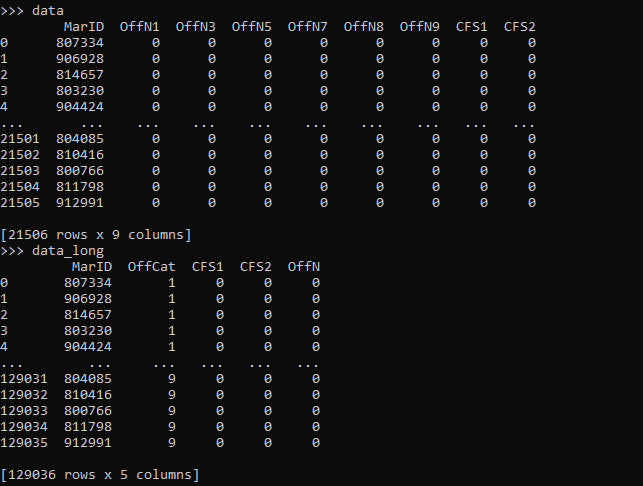For reference the offense categories are below, and I drop homicide/arson/sex abuse due to very low counts.

``````'''
Offense types in the data
OffN2   Arson #drop
OffN3   Burglary
OffN4   Homicide #drop
OffN5   Robbery
OffN6   Sex Abuse #drop
OffN7   Stolen Auto
OffN8   Theft
OffN9   Theft from Auto
'''``````

Now we can fit our stacked negative binomial model. I am going right to negative binomial, since I know this data is overdispersed and Poisson is not a good fit. I account for the clustering induced by stacking the equations, although with such a large sample it should not be a big deal.

``````# Fit a model with clustered standard errors
covp = {'groups': data_long['MarID'],'df_correction':True}
nb_mod = smf.negativebinomial('OffN ~ C(OffCat) + CFS1:C(OffCat) - 1',data_long).fit(cov_type='cluster',cov_kwds=covp)
print(nb_mod.summary())``````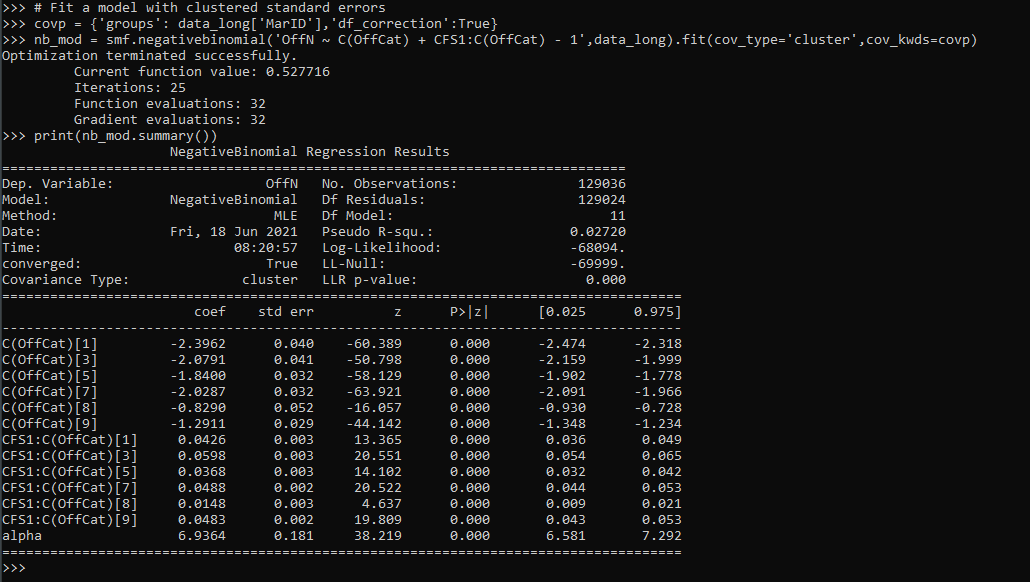So this is close to the same if you fit a separate regression for each crime type. You get an intercept for each crime type (the `C(OffCat[?])` coefficients), as well as a varying treatment effect for calls for service across each crime type, e.g. `CFS1:C(OffCat)` is the effect of 311 calls to increase Assaults, `CFS1:C(OffCat)` is to increase Burglaries, etc.

One limitation of this approach is that alpha here is constrained to be equal across each crime type. (Stata can get around this, either with the stacked equation fitting an equation for alpha based on the offense categories, or using the suest command.) But it is partly sweating the small stuff, the mean equation is the same. (So it may also make sense to not worry about clustering and fit a robust covariance estimate to the Poisson equation.)

Now onto the hypothesis tests. Besides seeing whether any individual coefficient equals 0, we may also have two additional tests. One is whether the treatment effect is equal across the different crime types. Here is how you do that in python for this example:

``````# Conduct a Wald test for equality of multiple coefficients
x_vars = nb_mod.summary2().tables.index
wald_str = ' = '.join(list(x_vars[6:-1]))
print(wald_str)
wald_test = nb_mod.wald_test(wald_str) # joint test
print(wald_test)``````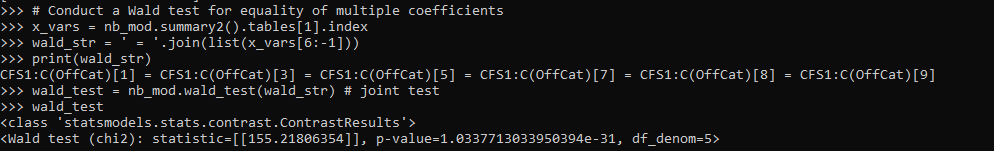Given the large sample size, even though all of the coefficients for garbage 311 calls are very similar (mostly around 0.3~0.5), this joint test says they do not all equal each other.

So the second hypothesis people are typically interested in is whether the coefficients all equal zero, a joint test. Here is how you do that in python statsmodels. They have a convenient function `.wald_test_terms()` to do just this, but I also show how to construct the same string and use `.wald_test()`.

``````# Or can do test all join equal 0
nb_mod.wald_test_terms()
# To replicate what wald_test_terms is doing yourself
all_zero = [x + '= 0' for x in x_vars[6:-1]]
nb_mod.wald_test(','.join(all_zero))``````

So we have established that when testing the equality between the coefficients, we reject the null. But this does not tell us which contrasts are themselves different and the magnitude of those coefficient differences. We can use .`t_test()` for that. (Which is the same as a Wald test, just looking at particular contrasts one by one.)

``````# Pairwise contrasts of coefficients
# To get the actual difference in coefficients
wald_li = []
for a,b in itertools.combinations(x_vars[6:-1],2):
wald_li.append(a + ' - ' + b + ' = 0')

wald_dif = ' , '.join(wald_li)

dif = nb_mod.t_test(wald_dif)
print(dif)

# c's correspond to the wald_li list
res_contrast = dif.summary_frame()
res_contrast['Test'] = wald_li
res_contrast.set_index('Test', inplace=True)
print(res_contrast)``````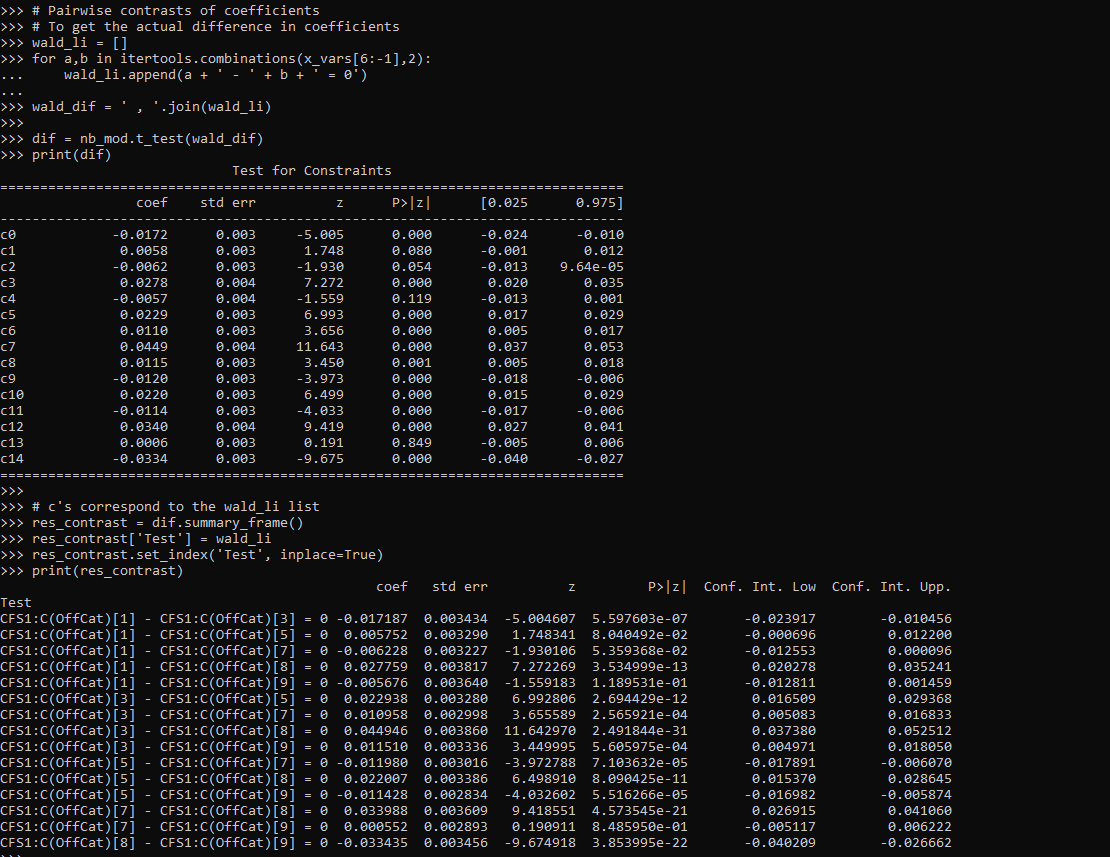You can see the original t-test table does not return a nice set of strings illustrating the actual test. So I show here how they correspond to a particular hypothesis. I actually wrote a function to give nice labels given an input test string (what you would submit to either `.t_test()` or `.wald_test()`).

``````# Nicer function to print out the actual tests it interprets as
# ends up being 1 - 3, 3 - 5, etc.
def nice_lab_tests(test_str,mod):
# Getting exogenous variables
x_vars = mod.summary2().tables.index
# Patsy getting design matrix and constraint from string
di = patsy.DesignInfo(x_vars)
const_mat = di.linear_constraint(test_str)
r_mat = const_mat.coefs
c_mat = list(const_mat.constants)
# Loop over the tests, get non-zero indices
# Build the interpreted tests
lab = []
for i,e in enumerate(c_mat):
lm = r_mat[i,:] #single row of R matrix
nz = np.nonzero(lm).tolist() #only need non-zero
c_vals = lm[nz].tolist()
v_labs = x_vars[nz].tolist()
fin_str = ''
in_val = 0
for c,v in zip(c_vals,v_labs):
# 1 and -1 drop values and only use +/-
if c == 1:
if in_val == 0:
fin_str += v
else:
fin_str += ' + ' + v
elif c == -1:
if in_val == 0:
fin_str += '-' + v
else:
fin_str += ' - ' + v
else:
if in_val == 0:
fin_str += str(c) + '*' + v
else:
if c > 0:
sg = ' + '
else:
sg = ' - '
fin_str += sg + str(np.abs(c)) + '*' + v
in_val += 1
fin_str += ' = ' + str(e) #set equality at end
lab.append(fin_str)
return lab``````

So if we look at our original `wald_str`, this converts the equality tests into a series of difference tests against zero.

``````# Wald string for equality across coefficients
# from earlier
lab_tests = nice_lab_tests(wald_str,nb_mod)
print(lab_tests)``````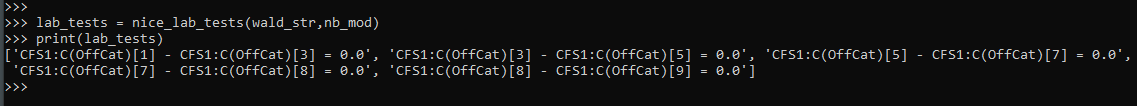And this function should work for other inputs, here is another example:

``````# Additional test to show how nice_lab_tests function works
str2 = 'CFS1:C(OffCat) = 3, CFS1:C(OffCat) = CFS1:C(OffCat)'
nice_lab_tests(str2,nb_mod)``````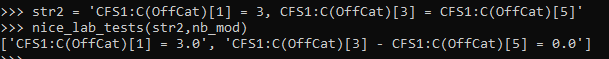Next up on the agenda is a need to figure out `.get_margeff()` a bit better for these statsmodels (or perhaps write my own closer to Stata’s implementation).

# Transforming predicted variables in regression

The other day on LinkedIn I made a point about how I think scikits `TransformedTargetRegressor` is very likely to mislead folks. In fact, the example use case in the docs for this function is a common mistake, fitting a model for `log(y)`, then getting predictions `phat`, and then simply exponentiating those predictions `exp(phat)`.

On LinkedIn I gave an example of how this is problematic for random forests, but here is a similar example for linear regression. For simplicity pretend we only have 3 potential residuals (all equally likely), either a residual of -1, 0, or 1.

Now pretend our logged prediction is 5, so if we simply do `exp(5)` we get about `148`. Now what are our predictions is we consider those 3 potential residuals?

``````Resid  Pred-Resid Modified_Pred LinPred
-1     5 - -1        exp(6)     403
0     5 -  0        exp(5)     148
1     5 -  1        exp(4)      55``````

So if we take the mean of our `LinPred` column, we then get a prediction of about `202`. The prediction using this approach is much higher than the naive approach of simply exponentiating 5. The difference is that the exp(5) estimate is the median, and the above estimate taking into account residuals is the mean estimate.

While there are some cases you may want the median estimate, in that case it probably makes more sense to use a quantile estimator of the median from the get go, as opposed to doing the linear regression on `log(y)`. I think for many (probably most) use cases in which you are predicting dollar values, this underestimate can be very problematic. If you are using these estimates for revenue, you will be way under for example. If you are using these estimates for expenses, holy moly you will probably get fired.

This problem will happen for any non-linear transformation. So while some transformations are ok, in scikit for example minmax or standardnormal scalars are ok, things like logs, square roots, or box-cox transformations are not. (To know if it is a linear transformation, if you do a scatterplot of original vs transformed, if it is a straight line it is ok, if it is a curved line it is not!)

I had a friend go back and forth with me for a bit after I posted this. I want to be clear this is not me saying the model of `log(y)` is the wrong model, it is just to get the estimates for the mean predictions, you need to take a few steps. In particular, one approach to get the mean estimates is to use Duan’s Smearing estimator. I will show how to do that in python below using simulated data.

# Example Duan’s Smearing in python

So first, we import the libraries we will be using. And since this is simulated data, will be setting the seed as well.

``````######################################################
import pandas as pd
import numpy as np
np.random.seed(10)

from sklearn.linear_model import LinearRegression
from sklearn.compose import TransformedTargetRegressor
######################################################``````

Next I will create a simple linear model on the log scale. So the regression of the logged values is the correct one.

``````######################################################
# Make a fake dataset, say these are housing prices
n = (10000,1)
error = np.random.normal(0,1,n)
x1 = np.random.normal(10,3,n)
x2 = np.random.normal(5,1,n)
log_y = 10 + 0.2*x1 + 0.6*x2 + error
y = np.exp(log_y)

dat = pd.DataFrame(np.concatenate([y,x1,x2,log_y,error], axis=1),
columns=['y','x1','x2','log_y','error'])
x_vars = ['x1','x2']

# Lets look at a histogram of y vs log y
dat['y'].hist(bins=100)
dat['log_y'].hist(bins=100)
######################################################``````

Here is the histogram of the original values: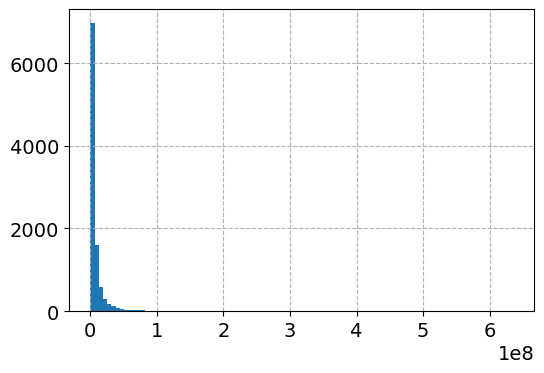And here is the histogram of the logged values: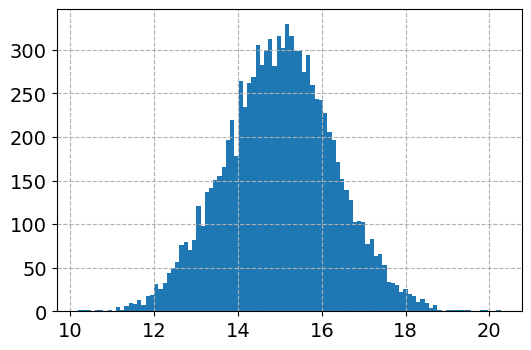So although the regression is the conditional relationship, if you see histograms like this I would also by default use a regression to predict `log(y)`.

Now here I do the same thing as in the original function docs, I fit a linear regression using the log as the function and exponential as the inverse function.

``````######################################################
# Now lets see what happens with the usual approach
tt = TransformedTargetRegressor(regressor=LinearRegression(),
func=np.log, inverse_func=np.exp)
tt.fit(dat[x_vars], dat['y'])
print( (tt.regressor_.intercept_, tt.regressor_.coef_) ) #Estimates the correct values

dat['WrongTrans'] = tt.predict(dat[x_vars])

dat[['y','WrongTrans']].describe()
######################################################``````

So here we estimate the correct simulated values for the regression equation: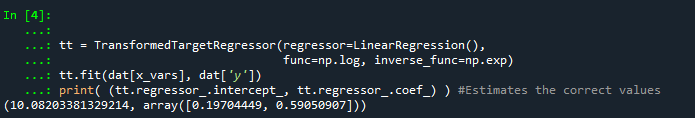But as we will see in a second, the exponentiated predictions are not so well behaved. To illustrate how the `WrongTrans` variable behaves, I show its distribution compared to the original `y` value. You can see that on average it is a much smaller estimate. Our sample values have a mean of 7.5 million, and the naive estimate here only has a mean of 4.6 million.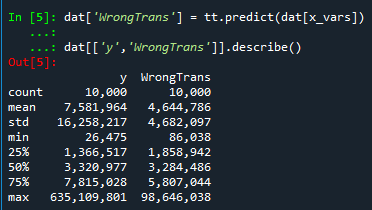Now here is a way to get an estimate of the mean value. In a nutshell, what you do is take the observed residuals, pretty much like that little table I did in the intro of this blog post, generate predictions given those residuals, and then back transform them and take the mean.

Although this example is using logged regression, I’ve made it pretty general. So if you used any box cox transformation instead of the logged (e.g. sklearns power_transform, it will work.

``````######################################################
# Duan's smearing, non-parametric approach via residuals

# Can make this general for any function inside of
# TransformedTargetRegressor
f = tt.get_params()['func']              #function
inv_f = tt.get_params()['inverse_func']  #and inverse function

# Non-parametric approach, approximate via residuals
log_pred = f(dat['WrongTrans'])
resids = f(dat['y']) - log_pred
resids = resids.values.reshape(1,n)
dp = inv_f(log_pred.values.reshape(n,1) + resids)
dat['DuanPreds'] = dp.mean(axis=1)

dat[['y','WrongTrans','DuanPreds']].describe()
######################################################``````

So you can see that the Duan Smeared predictions are looking better, at least the mean of the predictions is much closer to the original.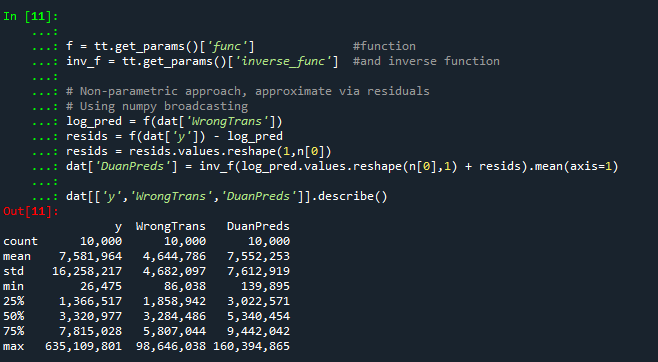I’ve intentionally done this example without using train/test, as we know the true answers. But in that case, you will want to use the residuals from the training dataset to apply this transformation to the test dataset.

So the residuals and the Duan smearing estimator do not need to be the same dimension. So for example if you have a big data application, you may want to do something like `resids = resids.sample(1000)` above.

Also another nice perk of this is you can use `dp` above to give you prediction intervals, so `np.quantile(dp,[0.025,0.975], axis=1).T` would give you a 95% prediction interval of the mean on the linear scale as well.

# Extra, Parametric Estimation

Another approach, which may make sense given the application, is instead of using the observed residuals to give a non-parametric estimate, you can estimate the distribution of the residuals, and then use that to make either an integral estimate of the Smeared estimate back on the original scale. Or in the case of the logged regression there is a closed form solution.

I show how to construct the integral estimator below, again trying to be more general. The integral approach will work for say any box-cox transformation.

``````######################################################
# Parametric approach, approximating residuals via normal

from scipy.stats import norm

# Look at the residuals again
resids = f(dat['y']) - f(tt.predict(dat[x_vars]))

# Check to make sure that the residuals are really close to normal
# Before doing this
resids.hist(bins=100)

# Fit to a normal distribution
loc, scale = norm.fit(resids)

# Define integral
def integrand(x,pred):
return norm.pdf(x, loc, scale)*inv_f(pred - x)

# Pred should be the logged prediction
# -50,50 should be changed if the residuals are scaled differently
def duan_param(pred):

# This takes awhile to apply to the whole data frame!
dat['log_pred'] = f(tt.predict(dat[x_vars]))
sub_dat['DuanParam'] = sub_dat['log_pred'].apply(duan_param)

# Can see that these are very similar to the non-parametric

And you can see that this normal based approximation works just fine here, since by construction the model residuals are pretty well behaved in my simulation.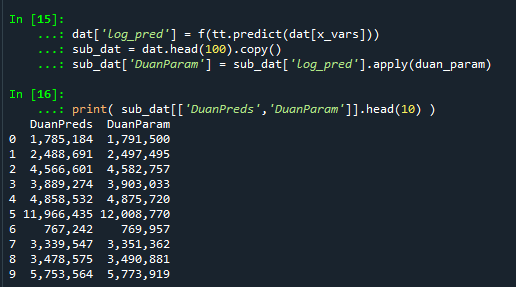It happens to be the case that there is a simpler estimate than the integral approach (which you can see in my notes takes awhile to estimate).

``````###########
# Easier way, but only applicable to log transform
# https://en.wikipedia.org/wiki/Smearing_retransformation
test_val = np.log(5000000)

# Integral approach
print( duan_param(test_val) )

# Approach for just log transformed
mult = np.exp(0.5*resids.var())
print( np.exp(test_val)*mult )
##########``````

So you can see the integral vs the closed form function are very close: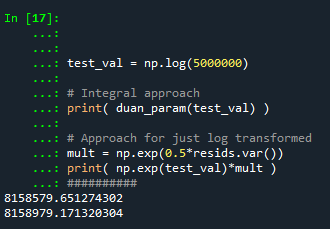The differences could be due to the the integral is simply an estimate (and you can see I did not do negative to positive infinity, but chopped it off, I do not know if there is a better function to estimate the integral or general approach here).

It wouldn’t surprise me if there are closed form solutions for box-cox transforms as well, but I am not familiar with them offhand. Again the integral approach (or the non-parametric approach) will work for whatever function you want. The function itself could be whatever crazy/discontinuous function you want. But this parametric Duan’s Smearing approach relies on the residuals being normally distributed. (I suppose you could use some other types of continuous distribution estimate if you have reason to, I have only seen normal distribution estimates though in practice.)

# Other Notes

While this focuses on regression, I do not think this will perform all that badly for other types of models (such as random forests or xgboost). But for forests it may make sense to simply pull out the individual tree estimates, back transform them, and get the mean of that backtransformed estimate. I have a different blog post that has a function showing how to scoop up the individual predictions from a random forest model.

It should also apply the same to any regression model with regularization. But if you want to do this, there are of course other alternative models you may consider that may be better suited towards your end goals of predictions on the linear/original scale.

For example, if you really want prediction intervals, it may make sense to not transform the data, and estimate a quantile regression model at the 5% and 95% quantiles. This would give you a 90% prediction interval.

Another approach is that it may make sense to use a different model, such as Poisson regression or negative binomial regression (or another generalized linear model in general). Even if your data are not integer counts, you can still use these models! (They just need to be 0 and above, no negative values.)

That Stata blog suggests to use Poisson and then robust standard errors, but that is a bad idea if you are really interested in predictions as well (see Gary Kings comment and linked paper). But you can just do negative binomial models in most cases then, and that is a better default than Poisson for many real world datasets.

# Making smoothed scatterplots in python

The other day I made a blog post on my notes on making scatterplots in matplotlib. One big chunk of why you want to make scatterplots though is if you are interested in a predictive relationship. Typically you want to look at the conditional value of the Y variable based on the X variable. Here are some example exploratory data analysis plots to accomplish that task in python.

I have posted the code to follow along on github here, in particular `smooth.py` has the functions of interest, and below I have various examples (that are saved in the `Examples_Conditional.py` file).

# Data Prep

First to get started, I am importing my libraries and loading up some of the data from my dissertation on crime in DC at street units. My functions are in the `smooth` set of code. Also I change the default matplotlib theme using `smooth.change_theme()`. Only difference from my prior posts is I don’t have gridlines by default here (they can be a bit busy).

``````#################################
import pandas as pd
import numpy as np
import matplotlib.pyplot as plt
import seaborn as sns
import statsmodels.api as sm
import os
import sys

mydir = r'D:\Dropbox\Dropbox\PublicCode_Git\Blog_Code\Python\Smooth'
data_loc = r'https://dl.dropbox.com/s/79ma3ldoup1bkw6/DC_CrimeData.csv?dl=0'
os.chdir(mydir)

#My functions
sys.path.append(mydir)
import smooth
smooth.change_theme()

#Dissertation dataset, can read from dropbox
#################################``````

# Binned Conditional Plots

The first set of examples, I bin the data and estimate the conditional means and standard deviations. So here in this example I estimate `E[Y | X = 0]`, `E[Y | X = 1]`, etc, where Y is the total number of part 1 crimes and x is the total number of alcohol licenses on the street unit (e.g. bars, liquor stores, or conv. stores that sell beer).

The function name is `mean_spike`, and you pass in at a minimum the dataframe, x variable, and y variable. I by default plot the spikes as +/- 2 standard deviations, but you can set it via the `mult` argument.

``````####################
#Example binning and making mean/std dev spike plots

smooth.mean_spike(DC_crime,'TotalLic','TotalCrime')

mean_lic = smooth.mean_spike(DC_crime,'TotalLic','TotalCrime',
plot=False,ret_data=True)
####################``````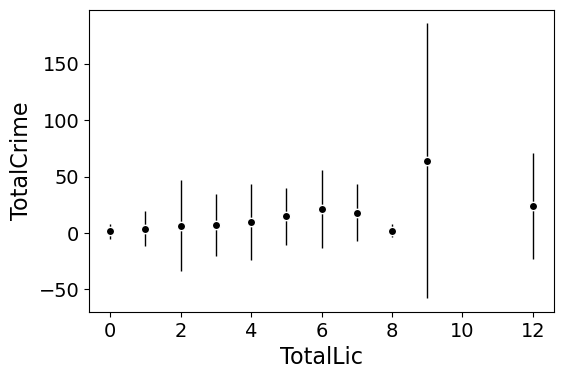This example works out because licenses are just whole numbers, so it can be binned. You can pass in any X variable that can be binned in the end. So you could pass in a string for the X variable. If you don’t like the resulting format of the plot though, you can just pass `plot=False,ret_data=True` for arguments, and you get the aggregated data that I use to build the plots in the end.

``````mean_lic = smooth.mean_spike(DC_crime,'TotalLic','TotalCrime',
plot=False,ret_data=True)``````

Another example I am frequently interested in is proportions and confidence intervals. Here it uses exact binomial confidence intervals at the 99% confidence level. Here I clip the burglary data to 0/1 values and then estimate proportions.

``````####################
#Example with proportion confidence interval spike plots

DC_crime['BurgClip'] = DC_crime['OffN3'].clip(0,1)
smooth.prop_spike(DC_crime,'TotalLic','BurgClip')

####################``````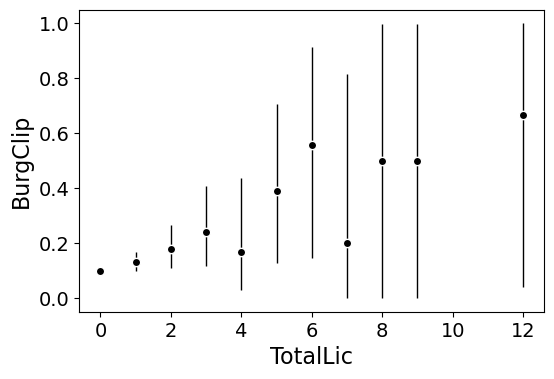A few things to note about this is I clip out bins with only 1 observation in them for both of these plots. I also do not have an argument to save the plot. This is because I typically only use these for exploratory data analysis, it is pretty rare I use these plots in a final presentation or paper.

I will need to update these in the future to jitter the data slightly to be able to superimpose the original data observations. The next plots are a bit easier to show that though.

# Restricted Cubic Spline Plots

Binning like I did prior works out well when you have only a few bins of data. If you have continuous inputs though it is tougher. In that case, typically what I want to do is estimate a functional relationship in a regression equation, e.g. `Y ~ f(x)`, where `f(x)` is pretty flexible to identify potential non-linear relationships.

Many analysts are taught the loess linear smoother for this. But I do not like loess very much, it is often both locally too wiggly and globally too smooth in my experience, and the weighting function has no really good default.

Another popular choice is to use generalized additive model smoothers. My experience with these (in R) is better than loess, but they IMO tend to be too aggressive, and identify overly complicated functions by default.

My favorite approach to this is actually then from Frank Harrell’s regression modeling strategies. Just pick a regular set of restricted cubic splines along your data. It is arbitrary where to set the knot locations for the splines, but my experience is they are very robust (so chaning the knot locations only tends to change the estimated function form by a tiny bit).

I have class notes on restricted cubic splines I think are a nice introduction. First, I am going to make the same dataset from my class notes, the US violent crime rate from 85 through 2010.

``````years = pd.Series(list(range(26)))
vcr = [1881.3,
1995.2,
2036.1,
2217.6,
2299.9,
2383.6,
2318.2,
2163.7,
2089.8,
1860.9,
1557.8,
1344.2,
1268.4,
1167.4,
1062.6,
945.2,
927.5,
789.6,
734.1,
687.4,
673.1,
637.9,
613.8,
580.3,
551.8,
593.1]

yr_df = pd.DataFrame(zip(years,years+1985,vcr), columns=['y1','years','vcr'])``````

I have a function that allows you to append the spline basis to a dataframe. If you don’t pass in a `data` argument, in returns a dataframe of the basis functions.

``````#Can append rcs basis to dataframe
kn = [3.0,7.0,12.0,21.0]
smooth.rcs(years,knots=kn,stub='S',data=yr_df)``````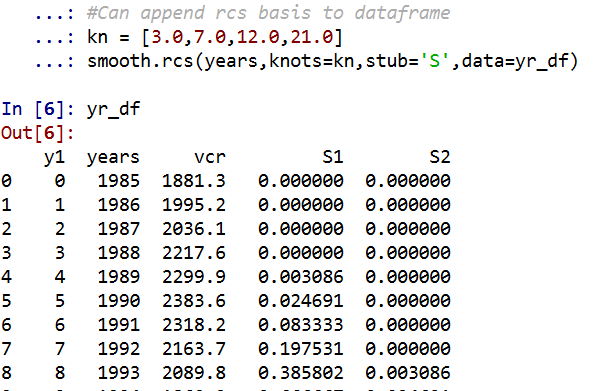I also have in the code set Harrell’s suggested knot locations for the data. This ranges from 3 to 7 knots (it will through an error if you pass a number not in that range). This here suggests the locations `[1.25, 8.75, 16.25, 23.75]`.

``````#If you want to use Harrell's rules to suggest knot locations
smooth.sug_knots(years,4)``````

Note if you have integer data here these rules don’t work out so well (can have redundant suggested knot locations). So Harell’s defaults don’t work with my alcohol license data. But it is one of the reasons I like these though, I just pick regular locations along the X data and they tend to work well. So here is a regression plot passing in those knot locations `kn = [3.0,7.0,12.0,21.0]` I defined a few paragraphs ago, and the plot does a few vertical guides to show the knot locations.

``````#RCS plot
smooth.plot_rcs(yr_df,'y1','vcr',knots=kn)``````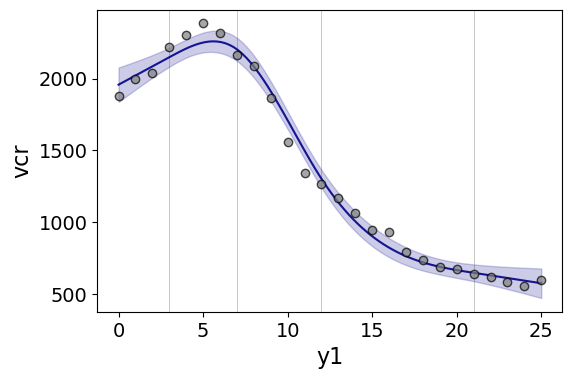Note that the error bands in the plot are confidence intervals around the mean, not prediction intervals. One of the nice things though about this under the hood, I used statsmodels glm interface, so if you want you can change the underlying link function to Poisson (I am going back to my DC crime data here), you just pass it in the `fam` argument:

``````#Can pass in a family argument for logit/Poisson models
smooth.plot_rcs(DC_crime,'TotalLic','TotalCrime', knots=[3,7,10,15],
fam=sm.families.Poisson(), marker_size=12)``````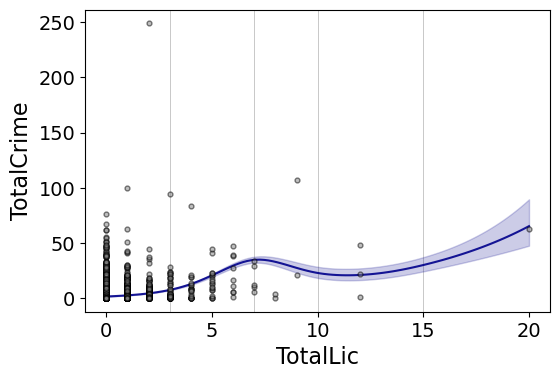This is a really great example for the utility of splines. I will show later, but a linear Poisson model for the alcohol license effect extrapolates very poorly and ends up being explosive. Here though the larger values the conditional effect fits right into the observed data. (And I swear I did not fiddle with the knot locations, there are just what I picked out offhand to spread them out on the X axis.)

And if you want to do a logistic regression:

``````smooth.plot_rcs(DC_crime,'TotalLic','BurgClip', knots=[3,7,10,15],
fam=sm.families.Binomial(),marker_alpha=0)``````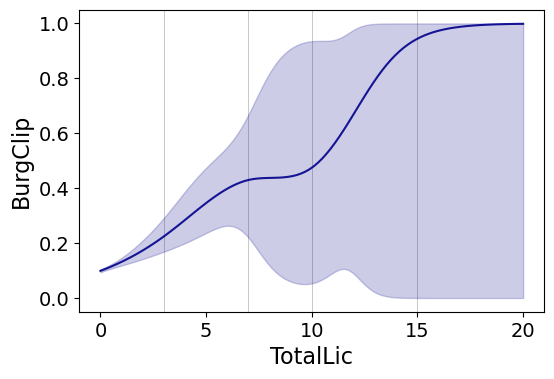I’m not sure how to do this in a way you can get prediction intervals (I know how to do it for Gaussian models, but not for the other glm families, prediction intervals probably don’t make sense for binomial data anyway). But one thing I could expand on in the future is to do quantile regression instead of glm models.

# Smooth Plots by Group

Sometimes you want to do the smoothed regression plots with interactions per groups. I have two helper functions to do this. One is `group_rcs_plot`. Here I use the good old iris data to illustrate, which I will explain why in a second.

``````#Superimposing rcs on the same plot
smooth.group_rcs_plot(iris,'sepal_length','sepal_width',
'species',colors=None,num_knots=3)``````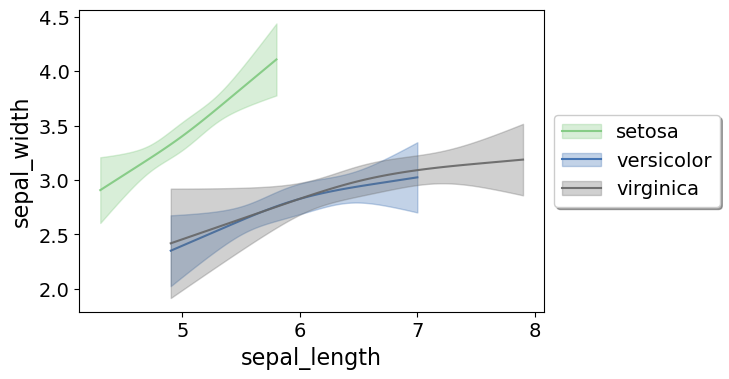If you pass in the `num_knots` argument, the knot locations are different for each subgroup of data (which I like as a default). If you pass in the `knots` argument and the locations, they are the same though for each subgroup.

Note that the way I estimate the models here I estimate three different models on the subsetted data frame, I do not estimate a stacked model with group interactions. So the error bands will be a bit wider than estimating the stacked model.

Sometimes superimposing many different groups is tough to visualize. So then a good option is to make a set of small multiple plots. To help with this, I’ve made a function `loc_error`, to pipe into seaborn’s small multiple set up:

``````#Small multiple example
g = sns.FacetGrid(iris, col='species',col_wrap=2)
g.map_dataframe(smooth.loc_error, x='sepal_length', y='sepal_width', num_knots=3)
g.set_axis_labels("Sepal Length", "Sepal Width")``````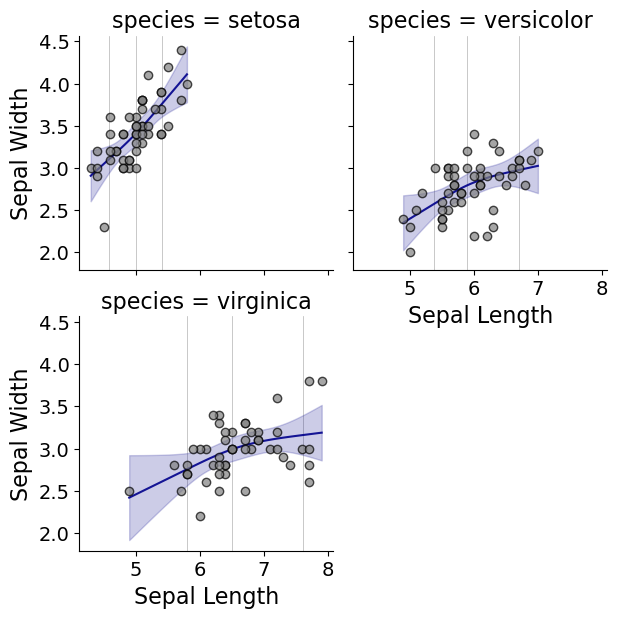And here you can see that the not locations are different for each subset, and this plot by default includes the original observations.

# Using the Formula Interface for Plots

Finally, I’ve been experimenting a bit with using the input in a formula interface, more similar to the way ggplot in R allows you to do this. So this is a new function, `plot_form`, and here is an example Poisson linear model:

``````smooth.plot_form(data=DC_crime,x='TotalLic',y='TotalCrime',
form='TotalCrime ~ TotalLic',
fam=sm.families.Poisson(), marker_size=12)``````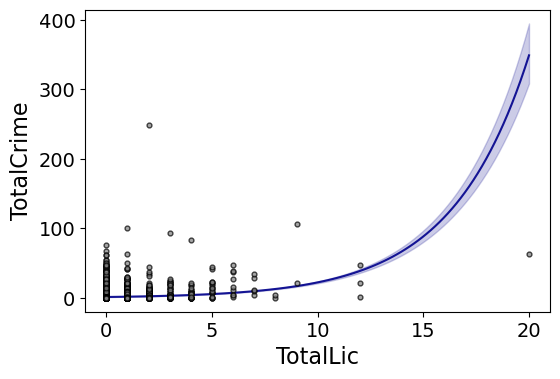You can see the explosive effect I talked about, which is common for Poisson/negative binomial models.

Here with the formula interface you can do other things, such as a polynomial regression:

``````#Can do polynomial terms
smooth.plot_form(data=DC_crime,x='TotalLic',y='TotalCrime',
form='TotalCrime ~ TotalLic + TotalLic**2 + TotalLic**3',
fam=sm.families.Poisson(), marker_size=12)``````

Which here ends up being almost indistinguishable from the linear terms. You can do other smoothers that are available in the patsy library as well, here are bsplines:

``````#Can do other smoothers
smooth.plot_form(data=DC_crime,x='TotalLic',y='TotalCrime',
form='TotalCrime ~ bs(TotalLic,df=4,degree=3)',
fam=sm.families.Poisson(), marker_size=12)``````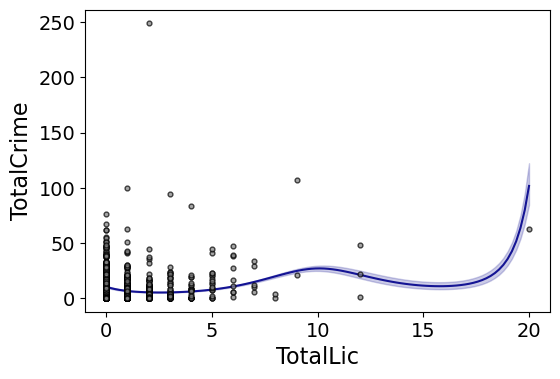I don’t really have a good reason to prefer restricted cubic splines to bsplines, I am just more familiar with restricted cubic splines (and this plot does not illustrate the knot locations that were by default chosen, although you could pass in knot locations to the `bs` function).

You can also do other transformations of the x variable. So here if you take the square root of the total number of licenses helps with the explosive effect somewhat:

``````#Can do transforms of the X variable
smooth.plot_form(data=DC_crime,x='TotalLic',y='TotalCrime',
form='TotalCrime ~ np.sqrt(TotalLic)',
fam=sm.families.Poisson(), marker_size=12)
``````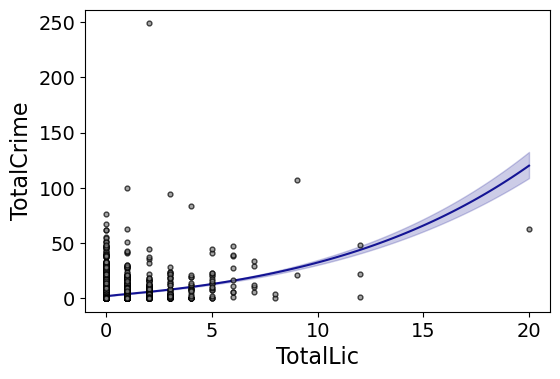In the prior blog post about explosive Poisson models I also showed a broken stick type model if you wanted to log the x variable but it has zero values.

``````#Can do multiple transforms of the X variable
smooth.plot_form(data=DC_crime,x='TotalLic',y='TotalCrime',
form='TotalCrime ~ np.log(TotalLic.clip(1)) + I(TotalLic==0)',
fam=sm.families.Poisson(), marker_size=12)``````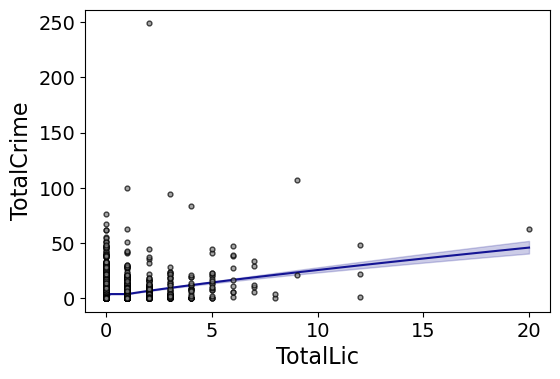Technically this “works” if you transform the Y variable as well, but the resulting plot is misleading, and the prediction interval is for the transformed variable. E.g. if you pass a formula `'np.log(TotalCrime+1) ~ TotalLic'`, you would need to exponentiate the the predictions and subtract 1 to get back to the original scale (and then the line won’t be the mean anymore, but the confidence intervals are OK).

I will need to see if I can figure out patsy and sympy to be able to do the inverse transformation to even do that. That type of transform to the y variable directly probably only makes sense for linear models, and then I would also maybe need to do a Duan type smearing estimate to get the mean effect right.

# Pooling multiple outcomes into one regression equation

Something that came up for many of my students this last semester in my Seminar in Research class is that many were interested in multiple outcomes. The most common one is examining different types of delinquency for juveniles (often via surveys), but it comes up in quite a few other designs as well (e.g. different crime outcomes for spatial research, different measures of perceptions towards police, different measures of fear of crime, etc.).

Most of the time students default to estimating separate equations for each of these outcomes, but in most circumstances I was telling the students they should pool these outcomes into one model. I think that is the better default for the majority of situations. So say we have a situation with two outcomes, violent crimes and property crimes, and we have one independent variable we are interested in, say whether an individual was subjected to a particular treatment. We might then estimate two separate equations:

``````E[# Violent Crimes]  = B0v + B1v*(Treatment)

E[# Property Crimes] = B0p + B1p*(Treatment)``````

By saying that I think by default we should think about pooling is basically saying that `B1v` is going to be close to equal to `B1p` in the two equations. Pooling the models together both lets us test that assertion, as well as get a better estimate of the overall treatment effect. So to pool the models we would stack the outcomes together, and then estimate something like:

``E[# Crimes (by type)] = B0 + B1*(Treatment) + B2*(Outcome = Violent) + B3(Treatment*Outcome = Violent)``

Here the B3 coefficient tests whether the treatment effect is different for the violent crime outcome as opposed to the property crime, and the dummy variable B2 effect controls for any differences in the levels of the two overall (that is, you would expect violent incidents to be less common than property crime incidents).

Because you will have multiple measures per individual, you can correct for that (by clustering the standard errors). But in the case you have many outcomes you might also want to consider a multi-level model, and actually estimate random effects for individuals and outcomes. So say instead of just violent and property crimes, but had a survey listing for 20 different types of delinquency. In that case you might want to do a model that looks like:

``Prob(Delinquency_ij) = f[B0 + B1*(Treatment_j) + d_j + g_i]``

Where one is estimating a multi-level logistic regression equation for delinquency type i within individual j, and the g_i and d_j are the random effects for delinquency types and individuals respectively. In the case you do not have many outcomes (say only 10), the random effect distribution might be hard to estimate. In that case I would just use fixed effects for the outcome dummy variables. But I can imagine the random effects for persons are of interest in many different study designs. And this way you get one model — instead of having to interpret 20+ models.

Also you can still estimate differential treatment effects across the different items if you want to, such as by looking at the interaction of the outcome types and the treatment. But in most cases in criminology I have come across treatments are general. That is, we would expect them to decrease/increase all crime types, not just some specific violent or property crime types. So to default pooling the treatment effect estimate makes sense.

To go a bit farther — juvenile delinquency is not my bag, but offhand I don’t understand why those who examine surveys of delinquency items use that multi-level model more often. Often times people aggregate the measures altogether into one overall scale, such as saying someone checked yes to 2 out of 10 violent crime outcomes, and checked yes to 5 out of 10 property crime outcomes. Analyzing those aggregated outcomes is another type of pooling, but one I don’t think is appropriate, mainly because it ignores the overall prevalence for the different items. For example, you might have an item such as "steal a car", and another that is "steal a candy bar". The latter is much more serious and subsequently less likely to occur. Going with my prior examples, pooling items together like this would force the random effects for the individual delinquency types, g_i, to all equal zero. Just looking at the data one can obviously tell that is not a good assumption.

Here I will provide an example via simulation to demonstrate this in Stata. First I generate an example dataset that has 1,000 individuals and 20 yes/no outcomes. They way the data are simulated is that each individual has a specific amount of `self_control` that decreases the probability of an outcome (with a coefficient of -0.5), they are nested within a particular group (imagine a different school) that affect whether the outcome occurs or not. In addition to this, each individual has a random intercept (drawn from a normal distribution), and each question has a fixed different prevalence.

``````*Stata simulation
clear
set more off
set seed 10
set obs 1000
generate caseid = _n
generate group = ceil(caseid/100)
generate self_control = rnormal(0,1)
generate rand_int = rnormal(0,1)

*generating 20 outcomes that just have a varying intercept for each
forval i = 1/20 {
generate logit_`i' = -0.4 -0.5*self_control -0.1*group + 0.1*(`i'-10) + rand_int
generate prob_`i' = 1/(1 + exp(-1*logit_`i'))
generate outcome_`i' = rbinomial(1,prob_`i')
}
drop logit_* prob_* rand_int
summarize prob_*``````

And here is that final output:

``````. summarize prob_*

Variable |        Obs        Mean    Std. Dev.       Min        Max
-------------+---------------------------------------------------------
prob_1 |      1,000    .1744795    .1516094   .0031003   .9194385
prob_2 |      1,000    .1868849     .157952   .0034252   .9265418
prob_3 |      1,000     .199886    .1642414   .0037841   .9330643
prob_4 |      1,000      .21348    .1704442   .0041804   .9390459
prob_5 |      1,000    .2276601    .1765258    .004618   .9445246
-------------+---------------------------------------------------------
prob_6 |      1,000     .242416    .1824513   .0051012   .9495374
prob_7 |      1,000    .2577337    .1881855   .0056347   .9541193
prob_8 |      1,000    .2735951    .1936933   .0062236   .9583033
prob_9 |      1,000     .289978    .1989401   .0068736    .962121
prob_10 |      1,000    .3068564    .2038919    .007591   .9656016
-------------+---------------------------------------------------------
prob_11 |      1,000    .3242004    .2085164   .0083827   .9687729
prob_12 |      1,000    .3419763    .2127823   .0092562   .9716603
prob_13 |      1,000    .3601469    .2166605   .0102197   .9742879
prob_14 |      1,000    .3786715    .2201237   .0112824   .9766776
prob_15 |      1,000    .3975066    .2231474   .0124542   .9788501
-------------+---------------------------------------------------------
prob_16 |      1,000    .4166057    .2257093    .013746   .9808242
prob_17 |      1,000    .4359203    .2277906   .0151697   .9826173
prob_18 |      1,000       .4554    .2293751   .0167384   .9842454
prob_19 |      1,000     .474993    .2304504   .0184663   .9857233
prob_20 |      1,000    .4946465    .2310073   .0203689   .9870643``````

You can see from this list that each `prob*` variable then has a different overall prevalence, from around 17% for prob_1, climbing to around 50% for prob_20.

Now if you wanted to pool the items into one overall delinquency scale, you might estimate a binomial regression model (note this is not a negative binomial model!) like below (see Britt et al., 2017 for discussion).

``````*first I will show the binomial model in Britt
egen delin_total = rowtotal(outcome_*)
*Model 1
glm delin_total self_control i.group, family(binomial 20) link(logit)``````

Which shows for the results (note that the effect of self-control is too small, it should be around -0.5):

``````. glm delin_total self_control i.group, family(binomial 20) link(logit)

Iteration 0:   log likelihood =  -3536.491
Iteration 1:   log likelihood = -3502.3107
Iteration 2:   log likelihood = -3502.2502
Iteration 3:   log likelihood = -3502.2502

Generalized linear models                         No. of obs      =      1,000
Optimization     : ML                             Residual df     =        989
Scale parameter =          1
Deviance         =  4072.410767                   (1/df) Deviance =   4.117706
Pearson          =  3825.491931                   (1/df) Pearson  =    3.86804

Variance function: V(u) = u*(1-u/20)              [Binomial]
Link function    : g(u) = ln(u/(20-u))            [Logit]

AIC             =     7.0265
Log likelihood   = -3502.250161                   BIC             =  -2759.359

------------------------------------------------------------------------------
|                 OIM
delin_total |      Coef.   Std. Err.      z    P>|z|     [95% Conf. Interval]
-------------+----------------------------------------------------------------
self_control |  -.3683605   .0156401   -23.55   0.000    -.3990146   -.3377065
|
group |
2  |   -.059046   .0666497    -0.89   0.376    -.1896769     .071585
3  |  -.0475712   .0665572    -0.71   0.475     -.178021    .0828785
4  |   .0522331   .0661806     0.79   0.430    -.0774786    .1819448
5  |  -.1266052   .0672107    -1.88   0.060    -.2583357    .0051254
6  |   -.391597   .0695105    -5.63   0.000     -.527835   -.2553589
7  |  -.2997012   .0677883    -4.42   0.000    -.4325639   -.1668386
8  |   -.267207   .0680807    -3.92   0.000    -.4006427   -.1337713
9  |  -.4340516   .0698711    -6.21   0.000    -.5709964   -.2971069
10  |  -.5695204    .070026    -8.13   0.000    -.7067689    -.432272
|
_cons |  -.5584345   .0470275   -11.87   0.000    -.6506067   -.4662623
------------------------------------------------------------------------------``````

One of the things I wish the Britt paper mentioned was that the above binomial model is equivalent to the a logistic regression model on the individual outcomes — but one that forces the predictions for each item to be the same across a person. So if you reshape the data from wide to long you can estimate that same binomial model as a logistic regression on the 0/1 outcomes.

``````*reshape wide to long
reshape long outcome_, i(caseid) j(question)
*see each person now has 20 questions each
*tab caseid

*regression model with the individual level data, should be equivalent to the aggregate binomial model
*Model 2
glm outcome_ self_control i.group, family(binomial) link(logit)``````

And here are the results:

``````. glm outcome_ self_control i.group, family(binomial) link(logit)

Iteration 0:   log likelihood = -12204.638
Iteration 1:   log likelihood = -12188.762
Iteration 2:   log likelihood = -12188.755
Iteration 3:   log likelihood = -12188.755

Generalized linear models                         No. of obs      =     20,000
Optimization     : ML                             Residual df     =     19,989
Scale parameter =          1
Deviance         =  24377.50934                   (1/df) Deviance =   1.219546
Pearson          =  19949.19243                   (1/df) Pearson  =   .9980085

Variance function: V(u) = u*(1-u)                 [Bernoulli]
Link function    : g(u) = ln(u/(1-u))             [Logit]

AIC             =   1.219975
Log likelihood   = -12188.75467                   BIC             =  -173583.3

------------------------------------------------------------------------------
|                 OIM
outcome_ |      Coef.   Std. Err.      z    P>|z|     [95% Conf. Interval]
-------------+----------------------------------------------------------------
self_control |  -.3683605   .0156401   -23.55   0.000    -.3990146   -.3377065
|
group |
2  |   -.059046   .0666497    -0.89   0.376    -.1896769     .071585
3  |  -.0475712   .0665572    -0.71   0.475     -.178021    .0828785
4  |   .0522331   .0661806     0.79   0.430    -.0774786    .1819448
5  |  -.1266052   .0672107    -1.88   0.060    -.2583357    .0051254
6  |   -.391597   .0695105    -5.63   0.000     -.527835   -.2553589
7  |  -.2997012   .0677883    -4.42   0.000    -.4325639   -.1668386
8  |   -.267207   .0680807    -3.92   0.000    -.4006427   -.1337713
9  |  -.4340516   .0698711    -6.21   0.000    -.5709964   -.2971069
10  |  -.5695204    .070026    -8.13   0.000    -.7067689    -.432272
|
_cons |  -.5584345   .0470275   -11.87   0.000    -.6506067   -.4662623
------------------------------------------------------------------------------``````

So you can see that Model 1 and Model 2 are exactly the same (in terms of estimates for the regression coefficients).

Model 2 though should show the limitations of using the binomial model — it predicts the same probability for each delinquency item, even though prob_1 is less likely to occur than prob_20. So for example, if we generate the predictions of this model, we can see that each question has the same predicted value.

``````predict prob_mod2, mu
sort question
by question: summarize outcome_ prob_mod2``````

And here are the results for the first four questions:

``````.     by question: summarize outcome_ prob_mod2

-------------------------------------------------------------------------------------------------------------------------------------------------------
-> question = 1

Variable |        Obs        Mean    Std. Dev.       Min        Max
-------------+---------------------------------------------------------
outcome_ |      1,000        .183      .38686          0          1
prob_mod2 |      1,000      .32305    .0924081   .1049203   .6537998

-------------------------------------------------------------------------------------------------------------------------------------------------------
-> question = 2

Variable |        Obs        Mean    Std. Dev.       Min        Max
-------------+---------------------------------------------------------
outcome_ |      1,000        .205    .4039036          0          1
prob_mod2 |      1,000      .32305    .0924081   .1049203   .6537998

-------------------------------------------------------------------------------------------------------------------------------------------------------
-> question = 3

Variable |        Obs        Mean    Std. Dev.       Min        Max
-------------+---------------------------------------------------------
outcome_ |      1,000        .208    .4060799          0          1
prob_mod2 |      1,000      .32305    .0924081   .1049203   .6537998

-------------------------------------------------------------------------------------------------------------------------------------------------------
-> question = 4

Variable |        Obs        Mean    Std. Dev.       Min        Max
-------------+---------------------------------------------------------
outcome_ |      1,000        .202    .4016931          0          1
prob_mod2 |      1,000      .32305    .0924081   .1049203   .6537998``````

By construction, the binomial model on the aggregated totals is a bad fit to the data. It predicts that each question should have a probability of around 32% of occurring. Although you can’t fit the zero-inflated model discussed by Britt via the individual level logit approach (that I am aware of), that approach has the same limitation as the generic binomial model approach. Modeling the individual items just makes more sense when you have the individual items. It is hard to think of examples where such a restriction would be reasonable for delinquency items.

So here a simple update is to include a dummy variable for each item. Here I also cluster according to whether the item is nested within an individual `caseid`.

``````*Model 3
glm outcome_ self_control i.group i.question, family(binomial) link(logit) cluster(caseid)``````

And here are the results:

``````.     glm outcome_ self_control i.group i.question, family(binomial) link(logit) cluster(caseid)

Iteration 0:   log pseudolikelihood = -11748.056
Iteration 1:   log pseudolikelihood = -11740.418
Iteration 2:   log pseudolikelihood = -11740.417
Iteration 3:   log pseudolikelihood = -11740.417

Generalized linear models                         No. of obs      =     20,000
Optimization     : ML                             Residual df     =     19,970
Scale parameter =          1
Deviance         =  23480.83406                   (1/df) Deviance =   1.175805
Pearson          =  19949.15609                   (1/df) Pearson  =   .9989562

Variance function: V(u) = u*(1-u)                 [Bernoulli]
Link function    : g(u) = ln(u/(1-u))             [Logit]

AIC             =   1.177042
Log pseudolikelihood = -11740.41703               BIC             =  -174291.8

(Std. Err. adjusted for 1,000 clusters in caseid)
------------------------------------------------------------------------------
|               Robust
outcome_ |      Coef.   Std. Err.      z    P>|z|     [95% Conf. Interval]
-------------+----------------------------------------------------------------
self_control |  -.3858319   .0334536   -11.53   0.000    -.4513996   -.3202641
|
group |
2  |  -.0620222   .1350231    -0.46   0.646    -.3266626    .2026182
3  |   -.049852   .1340801    -0.37   0.710    -.3126442    .2129403
4  |   .0549271   .1383412     0.40   0.691    -.2162167    .3260709
5  |  -.1329942   .1374758    -0.97   0.333    -.4024419    .1364535
6  |  -.4103578   .1401212    -2.93   0.003    -.6849904   -.1357253
7  |  -.3145033   .1452201    -2.17   0.030    -.5991296   -.0298771
8  |  -.2803599   .1367913    -2.05   0.040    -.5484659    -.012254
9  |  -.4543686   .1431314    -3.17   0.002    -.7349011   -.1738362
10  |  -.5962359   .1457941    -4.09   0.000    -.8819872   -.3104847
|
question |
2  |   .1453902   .1074383     1.35   0.176    -.0651851    .3559654
3  |   .1643203   .1094113     1.50   0.133     -.050122    .3787625
4  |   .1262597   .1077915     1.17   0.241    -.0850078    .3375272
5  |   .1830563    .105033     1.74   0.081    -.0228047    .3889173
6  |   .3609468   .1051123     3.43   0.001     .1549304    .5669633
7  |    .524749    .100128     5.24   0.000     .3285017    .7209963
8  |   .5768412   .1000354     5.77   0.000     .3807754     .772907
9  |   .7318797   .1021592     7.16   0.000     .5316513    .9321081
10  |    .571682   .1028169     5.56   0.000     .3701646    .7731994
11  |    .874362   .0998021     8.76   0.000     .6787535     1.06997
12  |   .8928982   .0998285     8.94   0.000     .6972379    1.088559
13  |   .8882734   .1023888     8.68   0.000      .687595    1.088952
14  |   .9887095   .0989047    10.00   0.000     .7948599    1.182559
15  |   1.165517   .0977542    11.92   0.000     .9739222    1.357111
16  |   1.230355   .0981687    12.53   0.000     1.037948    1.422762
17  |   1.260403   .0977022    12.90   0.000      1.06891    1.451896
18  |   1.286065    .098823    13.01   0.000     1.092376    1.479755
19  |   1.388013   .0987902    14.05   0.000     1.194388    1.581638
20  |   1.623689   .0999775    16.24   0.000     1.427737    1.819642
|
_cons |  -1.336376   .1231097   -10.86   0.000    -1.577666   -1.095085
------------------------------------------------------------------------------``````

You can now see that the predicted values for each individual item are much more reasonable. In fact they are a near perfect fit.

``````predict prob_mod3, mu
by question: summarize outcome_ prob_mod2 prob_mod3``````

And the results:

``````.     by question: summarize outcome_ prob_mod2 prob_mod3

-------------------------------------------------------------------------------------------------------------------------------------------------------
-> question = 1

Variable |        Obs        Mean    Std. Dev.       Min        Max
-------------+---------------------------------------------------------
outcome_ |      1,000        .183      .38686          0          1
prob_mod2 |      1,000      .32305    .0924081   .1049203   .6537998
prob_mod3 |      1,000        .183    .0672242   .0475809   .4785903

-------------------------------------------------------------------------------------------------------------------------------------------------------
-> question = 2

Variable |        Obs        Mean    Std. Dev.       Min        Max
-------------+---------------------------------------------------------
outcome_ |      1,000        .205    .4039036          0          1
prob_mod2 |      1,000      .32305    .0924081   .1049203   .6537998
prob_mod3 |      1,000        .205    .0729937   .0546202   .5149203

-------------------------------------------------------------------------------------------------------------------------------------------------------
-> question = 3

Variable |        Obs        Mean    Std. Dev.       Min        Max
-------------+---------------------------------------------------------
outcome_ |      1,000        .208    .4060799          0          1
prob_mod2 |      1,000      .32305    .0924081   .1049203   .6537998
prob_mod3 |      1,000        .208    .0737455    .055606   .5196471

-------------------------------------------------------------------------------------------------------------------------------------------------------
-> question = 4

Variable |        Obs        Mean    Std. Dev.       Min        Max
-------------+---------------------------------------------------------
outcome_ |      1,000        .202    .4016931          0          1
prob_mod2 |      1,000      .32305    .0924081   .1049203   .6537998
prob_mod3 |      1,000        .202    .0722336   .0536408   .5101407``````

If you want, you can also test whether any "treatment" effect (or here the level of a persons self control), has differential effects across the different delinquency items.

``````*Model 4
glm outcome_ self_control i.group i.question (c.self_control#i.question), family(binomial) link(logit) cluster(caseid)
*can do a test of all the interactions equal to zero at once
testparm c.self_control#i.question``````

I’ve omitted this output, but here of course the effect of self control is simulated to be the same across the different items, so one would fail to reject the null that any of the interaction terms are non-zero.

Given the way I simulated the data, the actual correct model is a random effects one. You should notice in each of the prior models the effect of self control is too small. One way to estimate that model in Stata is to below:

``````*Model 5
melogit outcome_ self_control i.group i.question || caseid:``````

And here are the results:

``````. melogit outcome_ self_control i.group i.question || caseid:

Fitting fixed-effects model:

Iteration 0:   log likelihood = -11748.056
Iteration 1:   log likelihood = -11740.418
Iteration 2:   log likelihood = -11740.417
Iteration 3:   log likelihood = -11740.417

Refining starting values:

Grid node 0:   log likelihood =  -10870.54

Fitting full model:

Iteration 0:   log likelihood =  -10870.54
Iteration 1:   log likelihood = -10846.176
Iteration 2:   log likelihood = -10845.969
Iteration 3:   log likelihood = -10845.969

Mixed-effects logistic regression               Number of obs     =     20,000
Group variable:          caseid                 Number of groups  =      1,000

Obs per group:
min =         20
avg =       20.0
max =         20

Integration method: mvaghermite                 Integration pts.  =          7

Wald chi2(29)     =    1155.07
Log likelihood = -10845.969                     Prob > chi2       =     0.0000
------------------------------------------------------------------------------
outcome_ |      Coef.   Std. Err.      z    P>|z|     [95% Conf. Interval]
-------------+----------------------------------------------------------------
self_control |  -.4744173   .0372779   -12.73   0.000    -.5474807    -.401354
|
group |
2  |  -.0648018   .1642767    -0.39   0.693    -.3867783    .2571746
3  |  -.0740465   .1647471    -0.45   0.653    -.3969449    .2488519
4  |    .036207   .1646275     0.22   0.826     -.286457     .358871
5  |  -.1305605   .1645812    -0.79   0.428    -.4531337    .1920126
6  |  -.5072909   .1671902    -3.03   0.002    -.8349776   -.1796042
7  |  -.3732567    .165486    -2.26   0.024    -.6976032   -.0489102
8  |  -.3495889   .1657804    -2.11   0.035    -.6745126   -.0246653
9  |  -.5593725   .1675276    -3.34   0.001    -.8877205   -.2310245
10  |  -.7329717   .1673639    -4.38   0.000    -1.060999   -.4049445
|
question |
2  |   .1690546   .1240697     1.36   0.173    -.0741177    .4122268
3  |    .191157   .1237894     1.54   0.123    -.0514657    .4337797
4  |   .1467393   .1243586     1.18   0.238    -.0969991    .3904776
5  |   .2130531   .1235171     1.72   0.085     -.029036    .4551422
6  |   .4219282   .1211838     3.48   0.000     .1844123    .6594441
7  |   .6157484   .1194133     5.16   0.000     .3817027    .8497941
8  |   .6776651   .1189213     5.70   0.000     .4445837    .9107465
9  |   .8626735   .1176486     7.33   0.000     .6320865    1.093261
10  |   .6715272   .1189685     5.64   0.000     .4383532    .9047012
11  |   1.033571   .1167196     8.86   0.000     .8048051    1.262338
12  |    1.05586    .116615     9.05   0.000     .8272985    1.284421
13  |   1.050297   .1166407     9.00   0.000     .8216858    1.278909
14  |   1.171248   .1161319    10.09   0.000     .9436331    1.398862
15  |   1.384883   .1154872    11.99   0.000     1.158532    1.611234
16  |   1.463414   .1153286    12.69   0.000     1.237375    1.689454
17  |   1.499836   .1152689    13.01   0.000     1.273913    1.725759
18  |   1.530954   .1152248    13.29   0.000     1.305117     1.75679
19  |   1.654674   .1151121    14.37   0.000     1.429058    1.880289
20  |   1.941035   .1152276    16.85   0.000     1.715193    2.166877
|
_cons |  -1.591796   .1459216   -10.91   0.000    -1.877797   -1.305795
-------------+----------------------------------------------------------------
caseid       |
var(_cons)|   1.052621   .0676116                      .9281064     1.19384
------------------------------------------------------------------------------
LR test vs. logistic model: chibar2(01) = 1788.90     Prob >= chibar2 = 0.0000``````

In that model it is the closest to estimating the correct effect of self control (-0.5). It is still small (at -0.47), but the estimate is within one standard error of the true value. (Another way to estimate this model is to use `xtlogit`, but with `melogit` you can actually extract the random effects. That will have to wait until another blog post though.)

Another way to think about this model is related to item-response theory, where individuals can have a latent estimate of how smart they are, and questions can have a latent easiness/hardness. In Stata you might fit that by the code below, but a warning it takes awhile to converge. (Not sure why, the fixed effects for questions are symmetric, so assuming a random effect distribution should not be too far off. If you have any thoughts as to why let me know!)

``````*Model 6
melogit outcome_ self_control i.group || caseid: || question:``````

For an academic reference to this approach see Osgood et al.,(2002). Long story short, model the individual items, but pool them together in one model!

# Don’t include temporal lags of crime in cross-sectional crime models

In my 311 and crime paper a reviewer requested I conduct cross-lagged models. That is, predict crime in 2011 while controlling for prior counts of crime in 2010, in addition to the other specific variables of interest (here 311 calls for service). In the supplementary material I detail why this is difficult with Poisson models, as the endogenous effect will often be explosive in Poisson models, something that does not happen as often in linear models.

There is a second problem though with cross-lagged models I don’t discuss though, and it has to do with how what I think a reasonable data generating process for crime at places can cause cross-lagged models to be biased. This is based on the fact that crime at places tends to be very temporally stable (see David Weisburd’s, or Martin Andresen’s, or my work showing that). So when you incorporate temporal lags of crime in models, this makes the other variables of interest (311 calls, alcohol outlets, other demographics, whatever) biased, because they cause crime in the prior time period. This is equivalent to controlling for an intermediate outcome. For examples of this see some of the prior work on the relationship between crime and disorder by Boggess and Maskaly (2014) or O’Brien and Sampson (2015).1

So Boggess and Maskaley (BM) and O’Brien and Sampson (OS) their simplified cross-lagged model is:

`(1) Crime_post = B0*Crime_pre + B1*physicaldisorder_pre`

Where the post and pre periods are yearly counts of crime and indicators of physical disorder. My paper subsequently does not include the prior counts of crime, but does lag the physical disorder measures by a year to ensure they are exogenous.

`(2) Crime_post = B1*physicaldisorder_pre`

There are a few reasons to do these lags. The most obvious is to make explanatory variable of broken windows exogenous, by making sure it is in the past. The reasons for including lags of crime counts are most often strictly as a control variable. There are some examples where crime begets more crime directly, such as retaliatory violence, (or see Rosenfeld, 2009) but most folks who do the cross-lagged models do not make this argument.

Now, my whole argument rests on what I think is an appropriate model explaining counts of crime at places. Continuing with the physical disorder example, I think a reasonable cross-sectional model of crime at places is that there are some underlying characteristics of locations that tend to be pretty stable over fairly long periods of time, and then we have more minor stuff like physical disorder that provide small exogenous shocks to the system over time.

`(3) Crime_i = B0*(physicaldisorder_i) + Z_i`

Where crime at location `i` is a function of some fixed characteristic `Z`. I can’t prove this model is correct, but I believe it is better supported by data. To support this position, I would refer to the incredibly high correlations between counts of crime at places from year to year. This is true of every crime dataset I have worked with (at every spatial unit of analysis), and is a main point of Shaw and McKay’s work plus Rob Sampsons for neighborhoods in Chicago, as well as David Weisburd’s work on trajectories of crime at street segments in Seattle. Again, this very high correlation doesn’t strike me as reasonably explained by crime causes more crime, what is more likely is that there are a set of fixed characteristics that impact criminal behavior at a certain locations.

If a model of crime is like that in (3), there are then two problems with the prior equations. The first problem for both (1) and (2) is that lagging physical disorder measures by a year does not make any sense. The idea behind physical disorder (a.k.a. broken windows) is that visible signs of disorder prime people to behave in a particular way. The priming presumably needs to be recent to affect behavior. But this can simply be solved by not lagging physical disorder by a year in the model. The lagged physical disorder effect might approximate the contemporaneous effect, if physical disorder itself is temporally consistent over long periods. So if say we replace physical disorder with locations of bars, the lagged effect of bars likely does not make any difference, between bars don’t turn over that much (and when they do they are oft just replaced by another bar).

But what if you still include the lags of crime counts? One may think that this controls for the omitted `Z_i` effect, but the effect is very bad for the other exogenous variables, especially lagged ones or temporally consistent ones. You are probably better off with the omitted random effect, because crime in the prior year is an intermediate outcome. I suspect this bias can be very large, and likely biases the effects of the other variables towards zero by quite alot. This is because effect of the fixed characteristic is large, the effect of the exogenous characteristic is smaller, and the two are likely correlated at least to a small amount.

To show this I conduct a simulation. SPSS Code here to replicate it. The true model I simulated is:

``````(4)  BW_it = 0.2*Z_i + ew_it
(5)  Crime_it = 5 + 0.1*BW_it + 0.9*Z_i + ec_it```````

I generated this for 25,000 locations and two time points (the t subscript), and all the variables are set to have a variance of 1 (all variables are normally distributed). The error terms (ew_it and ec_it) are not correlated, and are set to whatever value is necessary so the resultant variable on the left hand side has a variance of 1. With so many observations one simulation run is pretty representative of what would happen even if I replicated the simulation multiple times. This specification makes both BW (to stand for broken windows) and Z_i correlated.

In my run, what happens when we fit the cross-lagged model? The effect estimates are subsequently:

``````Lag BW:   -0.07
Lag Crime: 0.90``````

Yikes – effect of BW is in the opposite direction and nearly as large as the true effect. What about if you just include the lag of BW?

``Lag BW: 0.22``

The reason this is closer to the true effect is because of some round-about-luck. Since BW_it is correlated with the fixed effect Z_i, the lag of BW has a slight correlation to the future BW. This potentially changes how we view the effects of disorder on crime though. If BW is more variable, we can make a stronger argument that it is exogenous of other omitted variables. If it is temporally consistent it is harder to make that argument (it should also reduce the correlation with Z_i).

Still, the only reason this lag has a positive effect is that Z_i is omitted. For us to make the argument that this approximates the true effect, we have to make the argument the model has a very important omitted variable. Something one could only do as an act of cognitive dissonance.

How about use the contemporaneous effect of BW, but still include the lag counts of crime?

``````BW:        0.13
Lag Crime: 0.86``````

That is not as bad, because the lag of crime is now not an intermediate outcome. Again though, if we switch BW with something more consistent in time, like locations of bars, the lag will be an intermediate outcome, and will subsequently bias the effect. So what about a model of the contemporaneous effect of BW, omitting Z_i? The contemporaneous effect of BW will still be biased, since Z_i is omitted from the model.

``BW: 0.32``

But a way to reduce this bias is to introduce other control variables that approximate the omitted Z_i. Here I generate a set of 10 covariates that are a function of Z_i, but are otherwise not correlated with BW nor each other.

`(6) Oth_it = 0.5*Z_i + eoth_it`

Including these covariates in the model progressively reduces the bias. Here is a table for the reduction in the BW effect for the more of the covariates you add in, e.g. `with 2` means it includes two of the control variables in the model.

``````BW (with 0):  0.32
BW (with 1):  0.25
BW (with 2):  0.21
BW (with 3):  0.19
BW (with 10): 0.14``````

So if you include other cross-sectional covariates in an attempt to control for Z_i it brings the effect of BW closer to its true effect. This is what I believe happens in the majority of social science research that use strictly cross-sectional models, and is a partial defense of what people sometimes refer to kitchen sink models.

So in brief, I think using lags of explanatory variables and lags of crime in the same model are very bad, and can bias the effect estimates quite alot.

So using lags of explanatory variables and lags of crime counts in cross-sectional models I believe are a bad idea for most research designs. It is true that it makes it their effects exogenous, but it doesn’t eliminate the more contemporaneous effect of the variable, and so we may be underestimating the effect to a very large extent. Whether of not the temporal lag effects crime has to do with how the explanatory variable itself arises, and so the effect estimated by the temporal lag is likely to be misleading (and may be biased upward or downward depending on other parts of the model).

Incorporating prior crime counts is likely to introduce more bias than it solves I think for most cross-lagged models. I believe simply using a cross-sectional model with a reasonable set of control variables will get you closer to the real effect estimates than the cross-lagged models. If you think `Z_i` is correlated with a variable of interest (or lags of crime really do cause future crime) I think you need to do the extra step and have multiple time measures and fit a real panel data model, not just a cross lagged one.

I’m still not sure though when you are better off fitting a panel model versus expanding the time for the cross-section though. For one example, I think you are better off estimating the effects of demographic variables in a cross-sectional model, as opposed to a panel one, over a short period of time, (say less than 10 years). This is because demographic shifts simply don’t occur very fast, so there is little variance within units for a short panel.

1. I actually came up with the idea of using 311 calls independently of Dan O’Brien’s work, see my prospectus in 2013 in which I proposed the analysis. So I’m not totally crazy – although was alittle bummed to miss the timing abit! Four years between proposing and publishing the work is a bit depressing as well.

# Testing the equality of two regression coefficients

The default hypothesis tests that software spits out when you run a regression model is the null that the coefficient equals zero. Frequently there are other more interesting tests though, and this is one I’ve come across often — testing whether two coefficients are equal to one another. The big point to remember is that `Var(A-B) = Var(A) + Var(B) - 2*Cov(A,B)`. This formula gets you pretty far in statistics (and is one of the few I have memorized).

Note that this is not the same as testing whether one coefficient is statistically significant and the other is not. See this Andrew Gelman and Hal Stern article that makes this point. (The link is to a pre-print PDF, but the article was published in the American Statistician.) I will outline four different examples I see people make this particular mistake.

One is when people have different models, and they compare coefficients across them. For an example, say you have a base model predicting crime at the city level as a function of poverty, and then in a second model you include other control covariates on the right hand side. Let’s say the the first effect estimate of poverty is `3 (1)`, where the value in parentheses is the standard error, and the second estimate is `2 (2)`. The first effect is statistically significant, but the second is not. Do you conclude that the effect sizes are different between models though? The evidence for that is much less clear.

To construct the estimate of how much the effect declined, the decline would be `3 - 2 = 1`, a decrease in `1`. What is the standard error around that decrease though? We can use the formula for the variance of the differences that I noted before to construct it. So the standard error squared is the variance around the parameter estimate, so we have `sqrt(1^2 + 2^2) =~ 2.23` is the standard error of the difference — which assumes the covariance between the estimates is zero. So the standard error around our estimated decline is quite large, and we can’t be sure that it is an appreciably different estimate of poverty between the two models.

There are more complicated ways to measure moderation, but this ad-hoc approach can be easily applied as you read other peoples work. The assumption of zero covariance for parameter estimates is not a big of deal as it may seem. In large samples these tend to be very small, and they are frequently negative. So even though we know that assumption is wrong, just pretending it is zero is not a terrible folly.

The second is where you have models predicting different outcomes. So going with our same example, say you have a model predicting property crime and a model predicting violent crime. Again, I will often see people make an equivalent mistake to the moderator scenario, and say that the effect of poverty is larger for property than violent because one is statistically significant and the other is not.

In this case if you have the original data, you actually can estimate the covariance between those two coefficients. The simplest way is to estimate that covariance via seemingly unrelated regression. If you don’t though, such as when you are reading someone else’s paper, you can just assume the covariance is zero. Because the parameter estimates often have negative correlations, this assumption will make the standard error estimate smaller.

The third is where you have different subgroups in the data, and you examine the differences in coefficients. Say you had recidivism data for males and females, and you estimated an equation of the effect of a treatment on males and another model for females. So we have two models:

``````Model Males  : Prob(Recidivism) = B_0m + B_1m*Treatment
Model Females: Prob(Recidivism) = B_0f + B_1f*Treatment``````

Where the `B_0?` terms are the intercept, and the `B_1?` terms are the treatment effects. Here is another example where you can stack the data and estimate an interaction term to estimate the difference in the effects and its standard error. So we can estimate a combined model for both males and females as:

``Combined Model: Prob(Recidivism) = B_0c + B_1c*Treatment + B_2c*Female + B_3c(Female*Treatment)``

Where `Female` is a dummy variable equal to 1 for female observations, and `Female*Treatment` is the interaction term for the treatment variable and the Female dummy variable. Note that you can rewrite the model for males and females as:

``````Model Mal.: Prob(Recidivism) =     B_0c      +      B_1c    *Treatment    ....(when Female=0)
Model Fem.: Prob(Recidivism) = (B_0c + B_2c) + (B_1c + B_3c)*Treatment    ....(when Female=1)``````

So we can interpret the interaction term, `B_3c` as the different effect on females relative to males. The standard error of this interaction takes into account the covariance term, unlike estimating two totally separate equations would. (You can stack the property and violent crime outcomes I mentioned earlier in a synonymous way to the subgroup example.)

The final fourth example is the simplest; two regression coefficients in the same equation. One example is from my dissertation, the correlates of crime at small spatial units of analysis. I test whether different places that sell alcohol — such as liquor stores, bars, and gas stations — have the same effect on crime. For simplicity I will just test two effects, whether liquor stores have the same effect as on-premise alcohol outlets (this includes bars and restaurants). So lets say I estimate a Poisson regression equation as:

``log(E[Crime]) = Intercept + b1*Bars + b2*LiquorStores``

And then my software spits out:

``````                  B     SE
Liquor Stores    0.36  0.10
Bars             0.24  0.05``````

And then lets say we also have the variance-covariance matrix of the parameter estimates – which most stat software will return for you if you ask it:

``````                L       B
Liquor_Stores    0.01
Bars            -0.0002 0.0025``````

On the diagonal are the variances of the parameter estimates, which if you take the square root are equal to the reported standard errors in the first table. So the difference estimate is `0.36 - 0.24 = 0.12`, and the standard error of that difference is `sqrt(0.01 + 0.0025 - 2*-0.002) =~ 0.13`. So the difference is not statistically significant. You can take the ratio of the difference and its standard error, here `0.12/0.13`, and treat that as a test statistic from a normal distribution. So the rule that it needs to be plus or minus two to be stat. significant at the 0.05 level applies.

This is called a Wald test specifically. I will follow up with another blog post and some code examples on how to do these tests in SPSS and Stata. For completeness and just because, I also list two more ways to accomplish this test for the last example.

There are two alternative ways to do this test though. One is by doing a likelihood ratio test.

So we have the full model as:

`````` log(E[Crime]) = b0 + b1*Bars + b2*Liquor_Stores [Model 1]
``````

And we have the reduced model as:

`````` log(E[Crime]) = b4 + b5*(Bars + Liquor_Stores)  [Model 2]
``````

So we just estimate the full model with Bars and Liquor Stores on the right hand side (Model 1), then estimate the reduced model (2) with the sum of `Bars + Liquor Stores` on the right hand side. Then you can just do a chi-square test based on the change in the log-likelihood. In this case there is a change of one degree of freedom.

I give an example of doing this in R on crossvalidated. This test is nice because it extends to testing multiple coefficients, so if I wanted to test `bars=liquor stores=convenience stores`. The prior individual Wald tests are not as convenient for testing more than two coefficients equality at once.

Here is another way though to have the computer more easily spit out the Wald test for the difference between two coefficients in the same equation. So if we have the model (lack of intercept does not matter for discussion here):

``y = b1*X + b2*Z [eq. 1]``

We can test the null that b1 = b2 by rewriting our linear model as:

``y = B1*(X + Z) + B2*(X - Z) [eq. 2]``

And the test for the B2 coefficient is our test of interest The logic goes like this — we can expand `[eq. 2]` to be:

``y = B1*X + B1*Z + B2*X - B2*Z [eq. 3]``

which you can then regroup as:

``y = X*(B1 + B2) + Z*(B1 - B2) [eq. 4]``

and note the equalities between equations 4 and 1.

``B1 + B2 = b1; B1 - B2 = b2``

So B2 tests for the difference between the combined B1 coefficient. B2 is a little tricky to interpret in terms of effect size for how much larger b1 is than b2 – it is only half of the effect. An easier way to estimate that effect size though is to insert (X-Z)/2 into the right hand side, and the confidence interval for that will be the effect estimate for how much larger the effect of X is than Z.

Note that this gives an equivalent estimate as to conducting the Wald test by hand as I mentioned before.

# Regression to the mean – a tale of change scores

This is a real example from my work illustrating regression to the mean. I have a scale measuring impulsivity of offenders. I had an intervention that used cognitive behavioral thereapy (CBT) in a boot camp for one group, and business as usual for another (just plain old jail). I have measures of impulsivity at `pre`, `post`, and 6 month follow up (what I label as `post2`). CBT is suppossed to reduce impulsivity, and hopefully keep it that way.

I find that those who have gained the most during the intervention tend to revert back to their prior scores once they leave the bootcamp. That is, the measure `[post - pre]`, the gain in bootcamp, has a negative correlation with `[post2 - post]`, the loss after bootcamp. Is this due to the intervention being shitty? No! It is not — this is the result of regression to the mean. This does not show any relationship between the values, it will happen even if the impulse scores are totally random.

Note that the definition of covariance is:

``Cov(X,Y) = E[(x - E[X])*(y - E[Y])]``

Where `E` is representing the expectation, and `Cov(X,Y)` of course means the covariance between `X` and `Y`. Here for easier equations we can assume the mean in the impulse scale is zero across all three waves, which makes the means of the change scores zero as well (without any loss in generality). So dropping the inner expecations this equation reduces to:

``Cov(X,Y) = E[x*y]``

So defining `post-pre = Change1` and `post2 - post = Change2`, expanding out to the original components we have:

``Cov(Change1,Change2) = Cov(post-pre,post2-post) = E[ (post-pre)*(post2-post) ]``

The last result can then be expanded to:

``E[ post*post2 - post*post - pre*post2 + pre*post ]``

Because of the bilinearity of expectation, these can be further teased out:

``E[ post*post2 ] - E[ post*post ] - E[ pre*post2 ] + E[ pre*post]``

Note we can rewrite this back into variances and covariances of the original levels:

``Cov(post,post2) - Var(post) - Cov(pre,post2) + Cov(pre,post)``

There are two things to note here. 1) The covariances in the change scores can be entirely written as functions in the covariances of the levels. They do not supply information independent of the levels themselves.

For 2), if the data are random (that is the covariances between all the levels are random), the covariances between the change scores will be negative. This is because of the minus sign in front of the variance of the post term. For random data, all the other covariances are zero. This results in the correlation between the change scores being -1/2.

For a simple example in R:

``````> set.seed(10)
> n <- 10000 #sample size
> t1 <- rnorm(n) #three random vectors
> t2 <- rnorm(n)
> t3 <- rnorm(n)
> levels <- data.frame(t1,t2,t3)
> differ <- data.frame(c1=t2-t1,c2=t3-t2)
>
> #correlations in levels are approximately zero
> cor(levels)
t1           t2            t3
t1  1.0000000000  0.001874345 -0.0007006367
t2  0.0018743450  1.000000000 -0.0045967380
t3 -0.0007006367 -0.004596738  1.0000000000
>
> #correlation of differences is -0.5
> cor(differ)
c1         c2
c1  1.0000000 -0.4983006
c2 -0.4983006  1.0000000``````

Sometimes I see people talk about regression to the mean as if it is a sociological thing, like something that needs to be explained in terms of human behavior. It is not, it is entirely mathematical.

This is also one of the reasons I don’t like using change scores, either as independent or dependent variables. They typically can be rewritten in terms of the levels, and involve coeffficient restrictions that can have strange consequences. There are some situations (fixed effects) that make sense for the dependent variable. I haven’t seen a situation in the terms of independent variables where they make sense.

# Negative Binomial regression and predicted probabilities in SPSS

For my dissertation I have been estimating negative binomial regression models predicting the counts of crimes at small places (i.e. street segments and intersections). When evaluating the fit of poisson regression models and their variants, you typically make a line plot of the observed percent of integer values versus the predicted percent by the models. This is particularly pertinent for data that have a high proportion of zeros, as the negative binomial may still under-predict the number of zeros.

I mistakenly thought that to make such a plot you could simply estimate the predicted value following the negative binomial regression model and then round the predictions. But I was incorrect, and to make the typical predicted versus observed plot you need to estimate the probability of an observation taking an integer value, and then take the mean of that probability over all the observations. That mean will subsequently be the predicted percent given the model. Fortunately I caught my mistake before I gave some talks on my work recently, and I will show how to make said calculations in SPSS. I have posted the data to replicate this work at this dropbox link, and so you can download the data and follow along.

First, I got some help on how to estimate the predicted probabilities via an answer to my question at CrossValidated. So that question lists the formula one needs to estimate the predicted probability for any integer value N after the negative binomial model. To calculate that value though we need to make some special SPSS functions, the factorial and the complete gamma function. Both have SPSS tech help pages showing how to calculate them.

For the factorial we can use a general relationship with the `LNGAMMA` function.

``````
DEFINE !FACT (!POSITIONAL = !ENCLOSE("(",")"))
( EXP(LNGAMMA((!1)+1)) )
!ENDDEFINE.
``````

And for the complete gamma function we can use a relationship to the CDF of the gamma function.

``````
DEFINE !GAMMAF (!POSITIONAL = !ENCLOSE("(",")"))
( EXP(-1)/(!1)/(CDF.GAMMA(1,(!1),1) - CDF.GAMMA(1,(!1)+1,1)) )
!ENDDEFINE.
``````

And given these two functions, we can create a macro that takes as parameters and returns the predicted probability we are interested in:

• `out` – new variable name for predicted probability of taking on that integer value
• `PredN` – the predicted mean of the variable conditional on the covariates
• `Disp` – estimate of the dispersion parameter
• `Int` – the integer value being predicted
``````
DEFINE !PredNB (Out = !TOKENS(1)
/PredN = !TOKENS(1)
/Disp = !TOKENS(1)
/Int = !TOKENS(1) )
COMPUTE #a = (!Disp)**(-1).
COMPUTE #mu = !PredN.
COMPUTE #Y = !Int.
COMPUTE #1 = (!GAMMAF(#Y + #a))/(!FACT(#Y)*!GAMMAF(#a)).
COMPUTE #2 = (#a/(#a+#mu))**#a.
COMPUTE #3 =  (#mu/(#a + #mu))**#Y.
COMPUTE !Out =  #1*#2*#3.
!ENDDEFINE.
``````

But to make our plot we want to estimate this predicted probability over a range of values, so I created a helper macro that instead of taking only one integer value, takes the end integer value and will calculate the predicted probability of zero through N.

``````
DEFINE !PredNBRange (Num = !TOKENS(1)
/Mean = !TOKENS(1)
/Disp = !TOKENS(1)
/Stub = !TOKENS(1) )
!DO !I = 0 !TO !Num
!LET !Base = !CONCAT(!Stub,!I)
!PredNB Out = !Base PredN = !Mean Disp = !Disp Int = !I.
!DOEND
!ENDDEFINE.
``````

The example data and code I have posted compares these values to the ones predicted from Stata, and shows my function agrees with Stata to about 7 decimal points. I won’t go through all of those commands here, but I will show how to make the predicted proportions plot after you have a vector of predicted probabilities (you can download all of the code and data and the link I reference prior in the post).

So lets say that you have a vector `NB0 TO NB8`, and these are the predicted probabilities of integer values 0 to 8 for the observations in your dataset. To subsequently get the mean of the predictions, you can use the `AGGREGATE` command. Having no variables specified on the `BREAK` subcommand tells SPSS to aggregate over all values in the dataset. Here I export the file to a new dataset named `PredNBAgg`.

``````
DATASET DECLARE PredNBAgg.
AGGREGATE OUTFILE='PredNBAgg'
/BREAK =
/NB0 TO NB8 = MEAN(NB0 TO NB8).
``````

Now to merge later on to the observed proportions, I will reshape the dataset so the mean values are all in the same column using `VARSTOCASES`. Here I also make a category for the predicted probability of being 9 or higher (which isn’t typical for these types of plots, but something I believe is useful).

``````
DATASET ACTIVATE PredNBAgg.
COMPUTE NB9_Plus = 1 - SUM(NB0 TO NB8).
VARSTOCASES /MAKE NBPred FROM NB0 TO NB9_Plus /INDEX Int.
COMPUTE Int = Int - 1. /*Index starts at 1 instead of 0 */.
``````

Now I reactivate my original dataset, here named `PredNegBin`, calculate the binned observed values (with observations 9 and larger recoded to just 9) and then aggregate those values.

``````
DATASET ACTIVATE PredNegBin.
RECODE TotalCrime (9 THRU HIGHEST = 9)(ELSE = COPY) INTO Int.
DATASET DECLARE PredObsAgg.
AGGREGATE OUTFILE='PredObsAgg'
/BREAK = Int
/TotalObs = N.
``````

To get the predicted proportions within each category, I need to do another aggregation to get the total number of observations, and then divide the totals of each integer value with the total number of observations.

``````
DATASET ACTIVATE PredObsAgg.
AGGREGATE OUTFILE = * MODE=ADDVARIABLES OVERWRITE=YES
/BREAK =
/TotalN=SUM(TotalObs).
COMPUTE PercObs = TotalObs / TotalN.
``````

Now we can go ahead and merge the two aggregated datasets together. I also go ahead and close the old `PredNBAgg` dataset and define a value label so I know that the 9 integer category is really 9 and larger.

``````
MATCH FILES FILE = *
/FILE = 'PredNBAgg'
/BY Int.
DATASET CLOSE PredNBAgg.
VALUE LABELS Int 9 '9+'.
``````

Now at this point you could make the plot with the predicted and observed proportions in seperate variables, but this would take two `ELEMENT` statements within a `GGRAPH` command (and I like to make line plots with both the lines and points, so it would actually take 4 `ELEMENT` statements). So what I do here is reshape the data one more time with `VARSTOCASES`, and make a categorical variable to identify if the proportion is the observed value or the predicted value from the model. Then you can make your chart.

``````
VARSTOCASES /MAKE Dens FROM PercObs NBPred /Index Type /DROP TotalObs TotalN.
VALUE LABELS Type
1 'Observed'
2 'Predicted'.
GGRAPH
/GRAPHDATASET NAME="graphdataset" VARIABLES=Int Dens Type
/GRAPHSPEC SOURCE=INLINE.
BEGIN GPL
SOURCE: s=userSource(id("graphdataset"))
DATA: Int=col(source(s), name("Int"), unit.category())
DATA: Type=col(source(s), name("Type"), unit.category())
DATA: Dens=col(source(s), name("Dens"))
GUIDE: axis(dim(1), label("Total Crimes on Street Units"))
GUIDE: axis(dim(2), label("Percent of Streets"))
GUIDE: legend(aesthetic(aesthetic.color.interior), null())
SCALE: cat(aesthetic(aesthetic.color.interior), map(("1",color.black),("2",color.red)))
ELEMENT: line(position(Int*Dens), color.interior(Type))
ELEMENT: point(position(Int*Dens), color.interior(Type), color.exterior(color.white), size(size."7"))
END GPL.
``````

And voila, here you can see the predicted values are so close to the observed that it is difficult to even see the observed values. Here instead of creating a legend I manually added labels to the chart. A better chart may be to subtract the observed from predicted (especially if you were comparing multiple poisson models), but it should be quite plain to see that the negative binomial fits quite well to the observed data in this instance.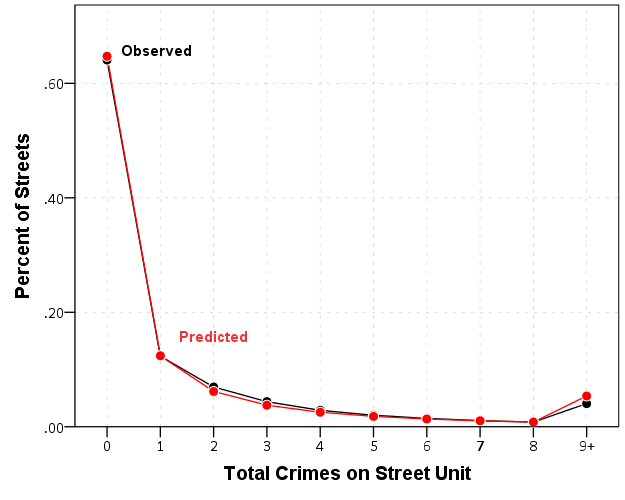Similar to Paul Allison’s experience, even with nearly 64% of the observations being zero, the negative binomial model fits just fine. I recently fit some other models with the same data (but a different outcome) in which the number of zeros were nearer to 90%. In that instance the negative binomial model would not converge, so estimating a zero inflated model was necessary. Here though it is clearly not necessary, and I would prefer the negative binomial model over a zip (or hurdle) as I see no obvious reason why I would prefer the complications of the different predicted zero equation in addition to the count equation.